# Mean-field stochastic linear quadratic optimal control problems: closed-loop solvability

## Abstract

An optimal control problem is studied for a linear mean-field stochastic differential equation with a quadratic cost functional. The coefficients and the weighting matrices in the cost functional are all assumed to be deterministic. Closed-loop strategies are introduced, which require to be independent of initial states; and such a nature makes it very useful and convenient in applications. In this paper, the existence of an optimal closed-loop strategy for the system (also called the closed-loop solvability of the problem) is characterized by the existence of a regular solution to the coupled two (generalized) Riccati equations, together with some constraints on the adapted solution to a linear backward stochastic differential equation and a linear terminal value problem of an ordinary differential equation.

## Introduction

Let $$(\Omega,{\mathcal {F}},\mathbb {F},\mathbb {P})$$ be a complete filtered probability space on which a standard one-dimensional Brownian motion W={W(t);0≤t<} is defined, where $$\mathbb {F}=\{{\mathcal {F}}_{t}\}_{t\geqslant 0}$$ is the natural filtration of W augmented by all the $$\mathbb {P}$$-null sets in $${\mathcal {F}}$$. Consider the following controlled linear mean-field stochastic differential equation (MF-SDE, for short) on a finite time horizon [t,T]:

{} \begin{aligned} \left\{\!\! \begin{array}{ll} dX(s)&\!\,=\,\left\{A(s)X(s)+\bar{A}(s)\mathbb{E}[X(s)]+B(s)u(s)+\bar{B}(s)\mathbb{E}[u(s)]+b(s)\right\}ds\\ &\quad+\!\left\{\!C(s)X(s)+\bar{C}(s)\mathbb{E}[\!X(s)]+D(s)u(s)\,+\,\bar{D}(s)\mathbb{E}[u(s)]+\sigma(s)\right\}\!dW(s),\quad s\in[t,T],\\ X(t)&\!\,=\,\xi, \end{array} \right. \end{aligned}
(1.1)

where $$A(\cdot), \bar {A}(\cdot), B(\cdot), \bar {B}(\cdot), C(\cdot), \bar {C}(\cdot), D(\cdot), \bar {D}(\cdot)$$ are given deterministic matrix-valued functions; b(·),σ(·) are vector-valued $$\mathbb {F}$$-progressively measurable processes and ξ is an $${\mathcal {F}}_{t}$$-measurable random vector. In the above, u(·) is the control process and X(·) is the corresponding state process with initial pair (t,ξ). For any t[0,T), we define

\begin{aligned} {\mathcal{U}}[t,T]=\!\left\{\!u:[\!t,T]\!\times\Omega\to\!\mathbb{R}^{m}\!\left|\! \right. u(\cdot)~\text{is}~ \mathbb{F}\text{-progressively measurable}, \mathbb{E}{\int_{t}^{T}}\!|u(s)|^{2}ds\!<\!\infty\right\}. \end{aligned}

Any $$u(\cdot)\in {\mathcal {U}}[t,T]$$ is called an admissible control (on [t,T]). Under some mild conditions, for any initial pair (t,ξ) with $$\xi \in L^{2}_{{\mathcal {F}}_{t}}(\Omega ;\mathbb {R}^{n})$$ (the set of all $${\mathcal {F}}_{t}$$-measurable, square-integrable $$\mathbb {R}^{n}$$-valued processes), and any admissible control $$u(\cdot)\in {\mathcal {U}}[t,T]$$, (1.1) admits a unique square-integrable solution X(·)≡X(· ;t,ξ,u(·)). Now we introduce the following cost functional:

\begin{aligned} J(t,\xi;u(\cdot))&\triangleq\mathbb{E}\left\{\vphantom{\quad+2 \left. \left\langle\left(\begin{array}{ll} \bar{q}(s)\\ \bar{\rho}(s) \end{array} \right), \left.\left(\begin{array}{ll} \mathbb{E}[X(s)]\\ \mathbb{E}[u(s)] \end{array} \right) \right\rangle \right] ds \right.}\langle GX(T),X(T)\rangle+2\langle g,X(T)\rangle +\langle\bar{G}\mathbb{E}[X(T)],\mathbb{E}[X(T)]\rangle+2\langle\bar{g},\mathbb{E}[X(T)]\rangle\right. \\ &\quad+{\int_{t}^{T}} \left[ \left\langle\left(\begin{array}{ll}Q(s)&S(s)^{\top}\\ S(s)&R(s) \end{array} \right) \right. \left(\begin{array}{ll }X(s)\\ u(s)\end{array} \right), \left(\begin{array}{ll}X(s)\\ u(s) \end{array} \right) \right\rangle +2\left.\left\langle\left(\!\begin{array}{ll}q(s)\\ \rho(s)\end{array} \right), \left(\!\begin{array}{ll}X(s)\\ u(s) \end{array} \right) \right\rangle \right] ds \\ &\quad+{\int_{t}^{T}} \left[ \left\langle \left(\begin{array}{ll}\bar{Q}(s)&\bar{S}(s)^{\top}\\ \bar{S}(s)&\bar{R}(s) \end{array} \right) \right. \left(\begin{array}{ll} \mathbb{E}[X(s)]\\ \mathbb{E}[u(s)] \end{array} \right), \left(\begin{array}{ll} \mathbb{E}[X(s)]\\ \mathbb{E}[u(s)] \end{array} \right) \right\rangle \\ &\quad+2 \left. \left\langle\left(\begin{array}{ll} \bar{q}(s)\\ \bar{\rho}(s) \end{array} \right), \left.\left(\begin{array}{ll} \mathbb{E}[X(s)]\\ \mathbb{E}[u(s)] \end{array} \right) \right\rangle \right] ds \right\},~~~~~~ \end{aligned}
(1.2)

where G, $$\bar {G}$$ are symmetric matrices and $$Q(\cdot), \bar {Q}(\cdot), S(\cdot), \bar {S}(\cdot), R(\cdot), \bar {R}(\cdot)$$ are deterministic matrix-valued functions with Q(·), $$\bar {Q}(\cdot)$$, R(·), and $$\bar {R}(\cdot)$$ being symmetric; g is an $${\mathcal {F}}_{t}$$-measurable random vector and $$\bar {G}$$ is a deterministic vector; q(·), ρ(·) are vector-valued $$\mathbb {F}$$-progressively measurable processes and $$\bar {Q}(\cdot)$$, $$\bar {\rho }(\cdot)$$ are vector-valued deterministic functions. Our mean-field stochastic linear quadratic (LQ, for short) optimal control problem can be stated as follows:

Problem (MF-LQ). For any given initial pair $$(t,\xi)\in [0,T)\times L^{2}_{{\mathcal {F}}_{t}}(\Omega ;\mathbb {R}^{n})$$, find a $$u^{*}(\cdot)\in {\mathcal {U}}[t,T]$$ such that

$$J(t,\xi;u^{*}(\cdot))=\inf_{u(\cdot)\in{\mathcal{U}}[t,T]}J(t,\xi;u(\cdot))\triangleq V(t,\xi).$$
(1.3)

Any $$u^{*}(\cdot)\in {\mathcal {U}}[t,T]$$ satisfying (1.3) is called an optimal open-loop control of Problem (MF-LQ) for the initial pair (t,ξ), and the corresponding X (·)≡X(· ;t,ξ,u (·)) is called an optimal open-loop state process. The function V(·,·) is called the value function of Problem (MF-LQ). In the special case where b(·), σ(·), g, $$\bar g$$, q(·), $$\bar q(\cdot)$$, ρ(·), and $$\bar \rho (\cdot)$$ vanish, we denote the corresponding mean-field LQ problem, cost functional, and value function by Problem (MF-LQ)0, J 0(t,ξ;u(·)), and V 0(t,ξ), respectively.

The theory of MF-SDEs can be traced back to Kac who presented a stochastic toy model for the Vlasov kinetic equation of plasma in (Kac 1956) which leads to the so-called McKean-Vlasov stochastic differential equation. Since then, the research on the related topics and their applications has become a notable and serious endeavor among researchers in applied probability and optimal stochastic controls, including financial engineering. See, for examples, Andersson and Djehiche (2011), Buckdahn et al. (2009), Buckdahn et al. (2009), Buckdahn et al. (2011), Cui et al. (2014), Elliott et al. (2013), Huang et al. (2015), Huang et al. (2015), McKean (1966), Meyer-Brandis et al. (2012), Yong (2013). Note that when the mean-field part is absent, Problem (MF-LQ) is reduced to the classical stochastic LQ optimal control problem. For relevant results and historic remarks on this subject, the reader is further referred to, for examples, (Ait Rami et al. 2001; Chen and Yong 2000; Chen et al. 1998; Tang 2003; Wonham 1968) and the book of Yong and Zhou (1999).

Recently, Sun and Yong introduced the notions of open-loop and closed-loop solvabilities for stochastic LQ problems (Sun and Yong 2014). See also (Sun et al. 2016; Sun et al. 2015). It turns out that these two notions are essentially different for stochastic LQ problem on finite time horizon. Roughly speaking, the open-loop solvability is equivalent to the solvability of a forward-backward stochastic differential equation (FBSDE, for short), and the closed-loop solvability is equivalent to the existence of a regular solution to a Riccati equation. Open-loop solvability was studied for Problem (MF-LQ) in (Sun 2016). The current work is therefore a continuation of the above-mentioned works.

The rest of the paper is organized as follows. In Section “Preliminaries” we give some preliminaries, carefully explain the closed-loop strategies, and introduce the regular solution to the generalized Riccati equation. Section “Necessary Conditions for Closed-Loop Solvability” is devoted to the necessary conditions for the existence of an optimal closed-loop strategy. In Section “Characterization of Closed-Loop Solvability”, we present our main result, in which the closed-loop solvability of the mean-field LQ problems is characterized. Finally, some concluding remarks are given in Section “Conclusion”.

## Preliminaries

We begin with some notations that will be used throughout the paper:

• $$\mathbb {R}^{n\times m}$$: the Euclidean space of all n×m real matrices.

• $$\mathbb {R}^{n}=\mathbb {R}^{n\times 1}$$ and $$\mathbb {R}=\mathbb {R}^{1}$$.

• $$\mathbb {S}^{n}$$: the space of all symmetric n×n real matrices.

• M : the transpose of a matrix M.

• M : the Moore–Penrose pseudoinverse of a matrix M (Penrose 1955).

• tr(M): the sum of diagonal elements of a square matrix M.

• 〈·,·〉: the inner product on a Euclidean space given by 〈M,Ntr(M N).

• $$|M|\triangleq \sqrt {\text {tr}(MM^{\top })}$$: the Frobenius norm of a matrix M.

• $${\mathcal {R}}(M)$$: the range of a matrix M.

For $$M,N\in \mathbb {S}^{n}$$, we use the notation $$M\geqslant N$$ (respectively, M>N) to indicate that MN is positive semi-definite (respectively, positive definite). For any $$\mathbb {S}^{n}$$-valued measurable function F on [t,T], we write

• $$F\geqslant 0 \quad \Longleftrightarrow \quad F(s)\geqslant 0, \quad \text {a.e.}~s\in [t,T]$$,

• F>0 F(s)>0, a.e. s[t,T],

• $$F\gg 0 \quad \Longleftrightarrow \quad F(s)\geqslant \delta I, \quad \text {a.e.}~s\in [t,T],\text {for some}~\delta >0$$.

Let f(·) be a function from $$\mathbb {R}^{n\times m}$$ into $$\mathbb {R}$$. Recall that the gradient of f at X=(x ij ), denoted by $$\frac {{\partial } f(X)}{{\partial } X}$$, is an n×m matrix whose (i,j)-th entry is given by $$\left [{{\partial } f(X)\over {\partial } X}\right ]_{ij}={{\partial } f(X)\over {\partial } x_{ij}}$$. For matrices L, M, and N of proper dimensions, the following formulae hold:

$$\begin{array}{lll}&\frac{{\partial}}{{\partial} X}~\text{tr}(LXM)=L^{\top} M^{\top},\quad &\frac{{\partial}}{{\partial} X}~\text{tr}(X^{\top}LXM)=LXM+L^{\top} XM^{\top},\\ &\frac{{\partial}}{{\partial} X}~\text{tr}(LX^{\top} M)=ML, \quad &\frac{{\partial}}{{\partial} X}~\text{tr}(LXMX^{\top} N)=L^{\top}N^{\top}XM^{\top}+NLXM. \end{array}$$

For a Euclidean space $$\mathbb {H}$$, let $$L^{p}(t,T;\mathbb {H}) (1\leqslant p\leqslant \infty)$$ be the space of all $$\mathbb {H}$$-valued functions that are L p-integrable on [t,T], and let $$C([t,T];\mathbb {H})$$ be the space of all $$\mathbb {H}$$-valued continuous functions on [t,T]. We denote

\begin{aligned} \begin{array}{ll} L^{2}_{{\mathcal{F}}_{t}}(\Omega;\mathbb{H})=\left\{\xi:\Omega\to\mathbb{H}|~\xi~\text{is}~ {\mathcal{F}}_{t}\text{-measurable},~ \mathbb{E}|\xi|^{2}<\infty\right\},\\ L_{\mathbb{F}}^{2}(t,T;\mathbb{H})=\left\{\varphi:[t,T]\times\Omega\to\mathbb{H}|~\varphi(\cdot)\text{is}~ \mathbb{F}\text{-progressively measurable},\, \mathbb{E}~{\int^{T}_{t}}|\varphi(s)|^{2}ds<\infty\right\},\\ L_{\mathbb{F}}^{2}(\Omega;C([t,T];\mathbb{H}))=\left\{\varphi:[t,T]\times\Omega\to\mathbb{H}|~\varphi(\cdot)\,\,\text{is}~ \mathbb{F}\text{-adapted, continuous},\, \mathbb{E}\left(\sup\limits_{s\in[t,T]}|\varphi(s)|^{2}\right)<\infty\right\},\\ L^{2}_{\mathbb{F}}(\Omega;L^{1}(t,T;\mathbb{H}))=\left\{\varphi:[t,T]\times\Omega\to\mathbb{H}|~\varphi(\cdot)\,\,\text{is}~ \mathbb{F}\text{-progressively measurable},~ \mathbb{E}\left({\int_{t}^{T}}|\varphi(s)|ds\right)^{2}\!<\infty\right\}. \end{array} \end{aligned}

The following assumptions will be in force throughout this paper.

(H1) The coefficients of the state equation satisfy the following:

\begin{aligned} \left\{\begin{array}{ll} A(\cdot),\bar{A}(\cdot)\in L^{1}(0,T;\mathbb{R}^{n\times n}), \quad B(\cdot),\bar{B}(\cdot)\in L^{2}(0,T;\mathbb{R}^{n\times m}), \quad b(\cdot)\in L^{2}_{\mathbb{F}}(\Omega;L^{1}(0,T;\mathbb{R}^{n})),\\ C(\cdot),\bar C(\cdot)\in L^{2}(0,T;\mathbb{R}^{n\times n}), \quad D(\cdot),\bar D(\cdot)\in L^{\infty}(0,T;\mathbb{R}^{n\times m}), \quad \sigma(\cdot)\in L^{2}_{\mathbb{F}}(0,T;\mathbb{R}^{n}). \end{array}\right. \end{aligned}

(H2) The weighting coefficients in the cost functional satisfy the following:

\begin{aligned} \left\{\begin{array}{ll} Q(\cdot), \bar{Q}(\cdot)\in L^{1}(0,T;\mathbb{S}^{n}), \quad S(\cdot), \bar{S}(\cdot)\in L^{2}(0,T;\mathbb{R}^{m\times n}), \quad R(\cdot), \bar{R}(\cdot)\in L^{\infty}(0,T;\mathbb{S}^{m}),\\ g\in L^{2}_{{\mathcal{F}}_{T}}(\Omega;\mathbb{R}^{n}), \quad q(\cdot)\in L^{2}_{\mathbb{F}}(\Omega;L^{1}(0,T;\mathbb{R}^{n})), \quad \rho(\cdot)\in L_{\mathbb{F}}^{2}(0,T;\mathbb{R}^{m}),\\ \bar{g}\in \mathbb{R}^{n},\quad \bar{q}(\cdot)\in L^{1}(0,T;\mathbb{R}^{n}), \quad \bar{\rho}(\cdot)\in L^{2}(0,T;\mathbb{R}^{m}),\quad G, \bar{G}\in\mathbb{S}^{n}. \end{array}\right. \end{aligned}

A standard argument using the contraction mapping theorem shows that under (H1), for any initial pair $$(t,\xi)\in [0,T)\times L^{2}_{{\mathcal {F}}_{t}}(\Omega ;\mathbb {R}^{n})$$ and any admissible control $$u(\cdot)\in {\mathcal {U}}[t,T]$$, (1.1) admits a unique (strong) solution $$X(\cdot)\equiv X(\cdot \,; t,\xi,u(\cdot))\in L_{\mathbb {F}}^{2}(\Omega ;C([t,T];\mathbb {R}^{n}))$$. Hence, under (H1)–(H2), the cost functional (1.2) is well-defined, and Problem (MF-LQ) makes sense.

Let us now recall the notion of open-loop solvability from Sun (2016), which was inspired by (Sun and Yong 2014).

### Definition 2.1

Problem (MF-LQ) is said to be (uniquely) open-loop solvable at initial pair $$(t,\xi)\in [0,T]\times L^{2}_{{\mathcal {F}}_{t}}(\Omega ;\mathbb {R}^{n})$$ if there exists a (unique) $$u^{*}(\cdot)\in {\mathcal {U}}[t,T]$$ satisfying (1.3). Problem (MF-LQ) is said to be (uniquely) open-loop solvable at t if for any $$\xi \in L^{2}_{{\mathcal {F}}_{t}}(\Omega ;\mathbb {R}^{n})$$, there exists a (unique) $$u^{*}(\cdot)\in {\mathcal {U}}[t,T]$$ satisfying (1.3), and Problem (MF-LQ) is said to be (uniquely) open-loop solvable on [t,T) if it is (uniquely) open-loop solvable at all s[t,T).

Next, we introduce the following definition.

### Definition 2.2

(i) LetAny tripleis called a closed-loop strategy of Problem (MF-LQ) on [t,T].

(ii) For any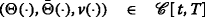and $$\xi \in L^{2}_{{\mathcal {F}}_{t}}(\Omega ;\mathbb {R}^{n})$$, let $$X(\cdot)\equiv X(\cdot \,;t,\xi,\Theta (\cdot),\bar \Theta (\cdot),v(\cdot))$$ be the solution to the following closed-loop system:

\begin{aligned} \left\{ \begin{array}{ll} dX(s)&=\left\{\left[A(s)+B(s)\Theta(s)\right]X(s)+\left\{\bar{A}(s)+B(s)\bar{\Theta}(s) +\bar{B}(s)\left[\Theta(s)+\bar{\Theta}(s)\right]\right\}\mathbb{E}[X(s)]\right.\\ &\qquad+\left.B(s)v(s)+\bar{B}(s)\mathbb{E}[v(s)]+b(s)\right\}ds\\ &+\left\{\left[C(s)+D(s)\Theta(s)\right]X(s)+\left\{\bar{C}(s)+D(s)\bar{\Theta}(s) +\bar{D}(s)\left[\Theta(s)+\bar{\Theta}(s)\right]\right\}\mathbb{E}[X(s)]\right.\\ &\qquad+D(s)v(s)+\left.\bar{D}(s)\mathbb{E}[v(s)]+\sigma(s)\right\} dW(s),\qquad s\in[t,T],\\ X(t)&=\xi, \end{array} \right. \end{aligned}
(2.1)

and let

$$u(s)=\Theta(s)X(s)+\bar{\Theta}(s)\mathbb{E}[X(s)]+v(s),\qquad s\in[t,T].$$

Then (X(·),u(·)) is called the outcome pair of $$(\Theta (\cdot),\bar {\Theta }(\cdot),v(\cdot))$$on [t,T] corresponding to the initial state ξ; X(·) and u(·) are called the corresponding closed-loop state process and closed-loop outcome control process, respectively.

Note that if (X(·),u(·)) is the outcome pair of a closed-loop strategycorresponding to some $$\xi \in L^{2}_{{\mathcal {F}}_{t}}(\Omega ;\mathbb {R}^{n})$$, then $$(X(\cdot),u(\cdot))\in L^{2}_{\mathbb {F}}(\Omega ;C([t,T];\mathbb {R}^{n})\times {\mathcal {U}}[t,T]$$ is actually a state-control pair of the state Eq. (1.1). In fact, (2.1) is equivalent to the following:

{} \begin{aligned} \left\{ \begin{array}{ll} dX(s)=\left\{A(s)X(s)+\bar A(s)\mathbb{E}[X(s)]+B(s)\left\{\Theta(s)X(s)+\bar\Theta(s)\mathbb{E}[X(s)]+v(s)\right\}\right.\\ \qquad\qquad\qquad~ +\left.\bar{B}(s)\mathbb{E}\left\{\Theta(s)X(s)+\bar{\Theta}(s)\mathbb{E}[X(s)]+v(s)\right\}+b(s)\right\}ds\\ \qquad\quad~~+\left\{C(s)X(s)+\bar C(s)\mathbb{E}[X(s)]+D(s)\left\{\Theta(s)X(s)+\bar{\Theta}(s)\mathbb{E}[X(s)]+v(s)\right\}\right.\\ \qquad\qquad\qquad~ +\left.\bar{D}(s)\mathbb{E}\left\{\Theta(s)X(s)+\bar\Theta(s)\mathbb{E}[X(s)]+v(s)\right\}+\sigma(s)\right\}dW(s),\quad s\in[t,T],\\ X(t)=\xi. \end{array}\right. \end{aligned}

Therefore, for any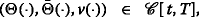, the meaning of $$J(t,\xi ;\Theta (\cdot)X(\cdot)+\bar {\Theta }(\cdot)\mathbb {E}[X(\cdot)]+v(\cdot))$$ is clear. We point out that a closed-loop strategy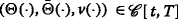is not related to any initial state $$\xi \in L^{2}_{{\mathcal {F}}_{t}}(\Omega ;\mathbb {R}^{n})$$, whereas, an outcome pair (X(·),u(·)) of a closed-loop strategy $$(\Theta (\cdot),\bar {\Theta }(\cdot),v(\cdot))$$ depends not only on the closed-loop strategy, but also on the initial state ξ. Hence, we should carefully distinguish the closed-loop strategy and the corresponding outcome control. Now, we are ready to introduce the following notion.

### Definition 2.3

(i) A closed-loop strategy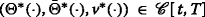is said to be optimal on [t,T] if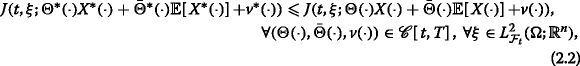where X (·) and X(·) are the closed-loop state processes corresponding to $$(\Theta ^{*}(\cdot),\bar {\Theta }^{*}(\cdot),v^{*}(\cdot),\xi)$$ and (Θ(·), $$\bar {\Theta }(\cdot),v(\cdot),\xi)$$, respectively. If an optimal closed-loop strategy (uniquely) exists on [t,T], Problem (MF-LQ) is said to be (uniquely) closed-loop solvable on [t,T].

(ii) A closed-loop strategyis said to be weakly optimal on [t,T] if (??) holds only for $$\xi =x\in \mathbb {R}^{n}$$. If a weakly optimal closed-loop strategy (uniquely) exists on [t,T], Problem (MF-LQ) is said to be (uniquely) weakly closed-loop solvable on [t,T].

Similar to (Sun and Yong 2014), we have the following proposition.

### Proposition 2.4

Let (H1)–(H2) hold and let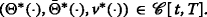. Then the following statements are equivalent:

(i) $$(\Theta ^{*}(\cdot),\bar {\Theta }^{*}(\cdot),v^{*}(\cdot))$$ is an optimal closed-loop strategy of Problem (MF-LQ) on [t,T];

(ii) The following holds:

\begin{aligned} \begin{array}{ll} J(t,\xi;\Theta^{*}(\cdot)X^{*}(\cdot)+\bar{\Theta}^{*}(\cdot)\mathbb{E}[X^{*}(\cdot)]+v^{*}(\cdot)) \leqslant J(t,\xi;\Theta^{*}(\cdot)X(\cdot)+\bar{\Theta}^{*}(\cdot)\mathbb{E}[X(\cdot)]+v(\cdot)),\\ \qquad\qquad\qquad\qquad\qquad\qquad\qquad\qquad\qquad\quad\forall(\xi,v(\cdot))\in L^{2}_{{\mathcal{F}}_{t}}(\Omega;\mathbb{R}^{n})\times{\mathcal{U}}[t,T], \end{array} \end{aligned}

where X (·) and X(·) are the closed-loop state processes corresponding to $$(\Theta ^{*}(\cdot),\bar {\Theta }^{*}(\cdot),v^{*}(\cdot),\xi)$$ and (Θ (·), $$\bar {\Theta }^{*}(\cdot),v(\cdot),\xi)$$, respectively;

(iii) The following holds:

\begin{aligned} J(t,\xi;\Theta^{*}(\cdot)X^{*}(\cdot)+\bar\Theta^{*}(\cdot)\mathbb{E}[\!X^{*}(\cdot)]+v^{*}(\cdot))\!\leqslant\! J(t,\xi;u(\cdot)),\quad\forall(\xi,u(\cdot))\!\in\! L^{2}_{{\mathcal{F}}_{t}}(\Omega;\mathbb{R}^{n})\times{\mathcal{U}}[\!t,T], \end{aligned}
(2.3)

where X (·)is the closed-loop state process corresponding to $$(\Theta ^{*}(\cdot),\bar \Theta ^{*}(\cdot),v^{*}(\cdot))$$ and $$\xi \in L^{2}_{{\mathcal {F}}_{t}}(\Omega ;\mathbb {R}^{n})$$.

### Proof.

(i) $$\mathop {\Rightarrow }$$ (ii) is trivial, by taking Θ(·)=Θ (·) and $$\bar {\Theta }(\cdot)=\bar {\Theta }^{*}(\cdot)$$ in (??).

(ii) $$\mathop {\Rightarrow }$$ (iii). For any $$\xi \in L^{2}_{{\mathcal {F}}_{t}}(\Omega ;\mathbb {R}^{n})$$ and $$u(\cdot)\in {\mathcal {U}}[t,T]$$, let X(·) be the solution to the following:

{} \begin{aligned}\left\{\! \begin{array}{ll} dX(s)\,=\,\left\{A(s)X(s)+\bar{A}(s)\mathbb{E}[X(s)]+B(s)u(s)+\bar{B}(s)\mathbb{E}[u(s)]+b(s)\right\}ds\\ \qquad\qquad+\!\left\{C(s)X(s)\,+\,\bar{C}(s)\mathbb{E}[\!X(s)]\!+D(s)u(s)\,+\,\bar{D}(s)\mathbb{E}[u(s)]+\sigma(s)\right\}\!dW(s),\quad\! s\in[t,T],\\ X(t)=\xi, \end{array} \right. \end{aligned}
(2.4)

and set

$$v(\cdot)\triangleq u(\cdot)-\Theta^{*}(\cdot)X(\cdot)-\bar{\Theta}^{*}(\cdot)\mathbb{E}[X(\cdot)]\in{\mathcal{U}}[t,T].$$

Then X(·) is also the solution to the following MF-SDE:

\begin{aligned} \left\{\! \begin{array}{ll} dX(s)\,=\,\left\{A(s)X(s)+\bar {A}(s)\mathbb{E}[X(s)]+B(s)\left\{\Theta^{*}(s)X(s)+\bar{\Theta}^{*}(s)\mathbb{E}[X(s)]+v(s)\right\}\right.\\ \qquad\qquad\qquad~+\left.\bar{B}(s)\mathbb{E}\left\{\Theta^{*}(s)X(s)+\bar{\Theta}^{*}(s)\mathbb{E}[X(s)]+v(s)\right\}+b(s)\right\}ds\\ \qquad\quad~~~+\left\{C(s)X(s)+\bar {C}(s)\mathbb{E}[X(s)]+D(s)\left\{\Theta^{*}(s)X(s)+\bar{\Theta}^{*}(s)\mathbb{E}[X(s)]+v(s)\right\}\right.\\ \qquad\qquad\qquad~+\left.\bar {D}(s)\mathbb{E}\left\{\!\Theta^{*}(s)X(s)+\bar\Theta^{*}(s)\mathbb{E}[\!X(s)]+v(s)\right\}+\sigma(s)\right\}dW(s),\quad s\in[t,T],\\ X(t)=\xi. \end{array}\right. \end{aligned}

Therefore,

$$\begin{array}{ll} J(t,\xi;\Theta^{*}(\cdot)X^{*}(\cdot)+\bar{\Theta}^{*}(\cdot)\mathbb{E}[X^{*}(\cdot)]+v^{*}(\cdot))\\ \leqslant J(t,\xi;\Theta^{*}(\cdot)X(\cdot)+\bar{\Theta}^{*}(\cdot)\mathbb{E}[X(\cdot)]+v(\cdot))=J(t,\xi;u(\cdot)). \end{array}$$

(iii) $$\mathop {\Rightarrow }$$ (i). For any $$\xi \in L^{2}_{{\mathcal {F}}_{t}}(\Omega ;\mathbb {R}^{n})$$ and, let X(·) be the solution to the following MF-SDE:

\begin{aligned} \left\{ \begin{array}{ll} dX(s)=\left\{A(s)X(s)+\bar A(s)\mathbb{E}[X(s)]+B(s)\left\{\Theta(s)X(s)+\bar{\Theta}(s)\mathbb{E}[X(s)]+v(s)\right\}\right.\\ \qquad\qquad\qquad~+\left.\bar{B}(s)\mathbb{E}\left\{\Theta(s)X(s)+\bar\Theta(s)\mathbb{E}[X(s)]+v(s)\right\}+b(s)\right\}ds\\ \qquad\quad~~~ +\left\{C(s)X(s)+\bar C(s)\mathbb{E}[X(s)]+D(s)\left\{\Theta(s)X(s)+\bar\Theta(s)\mathbb{E}[X(s)]+v(s)\right\}\right.\\ \qquad\qquad\qquad~+\left.\bar{D}(s)\mathbb{E}\left\{\Theta(s)X(s)+\bar{\Theta}(s)\mathbb{E}[X(s)]+v(s)\right\}+\sigma(s)\right\}dW(s),\quad s\in[t,T],\\ X(t)=\xi. \end{array}\right. \end{aligned}

Set

$$u(\cdot)\triangleq\Theta(\cdot)X(\cdot)+\bar{\Theta}(\cdot)\mathbb{E}[X(\cdot)]+v(\cdot)\in{\mathcal{U}}[t,T].$$

Then, by uniqueness, X(·) also solves MF-SDE (2.4). Thus,

$$\begin{array}{ll} J(t,\xi;\Theta^{*}(\cdot)X^{*}(\cdot)+\bar{\Theta}^{*}(\cdot)\mathbb{E}[X^{*}(\cdot)]+v^{*}(\cdot))\\ \leqslant J(t,\xi;u(\cdot))=J(t,\xi;\Theta(\cdot)X(\cdot)+\bar\Theta(\cdot)\mathbb{E}[X(\cdot)]+v(\cdot)). \end{array}$$

This completes the proof. □

With the same proof, we have the following result for the weakly optimal closed-loop strategies.

### Proposition 2.5

Let (H1)–(H2) hold and let. Then the following statements are equivalent:

(i) $$(\Theta ^{*}(\cdot),\bar {\Theta }^{*}(\cdot),v^{*}(\cdot))$$ is a weakly optimal closed-loop strategy of Problem (MF-LQ) on [t,T];

(ii) The following holds:

{} \begin{aligned} \begin{array}{ll} J(t,x;\Theta^{*}(\cdot)X^{*}(\cdot)+\bar{\Theta}^{*}(\cdot)\mathbb{E}[X^{*}(\cdot)]+v^{*}(\cdot)) \leqslant J(t,x;\Theta^{*}(\cdot)X(\cdot)+\bar{\Theta}^{*}(\cdot)\mathbb{E}[X(\cdot)]+v(\cdot)),\\ \qquad\qquad\qquad\qquad\qquad\qquad\qquad\qquad\qquad\qquad\qquad\quad\quad~\forall(x,v(\cdot))\in\mathbb{R}^{n}\times{\mathcal{U}}[\!t,T], \end{array} \end{aligned}

where X (·) and X(·) are the closed-loop state processes corresponding to $$(\Theta ^{*}(\cdot),\bar {\Theta }^{*}(\cdot),v^{*}(\cdot),x)$$ and (Θ (·), $$\bar {\Theta }^{*}(\cdot),v(\cdot),x)$$, respectively;

(iii) The following holds:

\begin{aligned} J(t,x;\Theta^{*}(\cdot)X^{*}(\cdot)+\bar{\Theta}^{*}(\cdot)\mathbb{E}[X^{*}(\cdot)]+v^{*}(\cdot)) \leqslant J(t,x;u(\cdot)),\!\quad\forall(x,u(\cdot))\in\mathbb{R}^{n}\times{\mathcal{U}}[t,T], \end{aligned}

where X (·) is the closed-loop state process corresponding to $$(\Theta ^{*}(\cdot),\bar {\Theta }^{*}(\cdot),v^{*}(\cdot))$$ and $$x\in \mathbb {R}^{n}$$.

### Remark 2.6

(i) An optimal open-loop control is allowed to depend on the initial state, whereas an optimal closed-loop strategy is required to be independent of the initial state.

(ii) It is clear from Proposition 2.4 (iii) that the outcome control $$u^{*}(\cdot)\equiv \Theta ^{*}(\cdot)X^{*}(\cdot)+\bar {\Theta }^{*}(\cdot)\mathbb {E}[X^{*}(\cdot)]+v^{*}(\cdot)$$ of an optimal closed-loop strategy $$(\Theta ^{*}(\cdot),\bar {\Theta }^{*}(\cdot),v^{*}(\cdot))$$ is an optimal open-loop control of Problem (MF-LQ) for the initial pair (t,X (t)). Hence, closed-loop solvability implies open-loop solvability.

(iii) Obviously, an optimal closed-loop strategy on [t,T]is also weakly optimal. For the classical LQ optimal control problems where $$\mathbb {E}[X(\cdot)]$$ and $$\mathbb {E}[u(\cdot)]$$ are absent, it can be shown, using the results from Sun and Yong ( 2014 ) and a completion of squares technique, that the two concepts coincide. But for Problem (MF-LQ), the existence of a weakly optimal closed-loop strategy does not guarantee the existence of an optimal closed-loop strategy. To see this, we present the following example.

### Example 2.7

Consider the following one-dimensional state equation

$$\left\{\begin{array}{ll} dX(s)=\left\{u(s)-\mathbb{E}[u(s)]\right\}ds+\mathbb{E}[u(s)]dW(s),\qquad s\in[t,1],\\ X(t)=\xi, \end{array}\right.$$
(2.5)

and cost functional

$$J(t,\xi;u(\cdot))=\mathbb{E}\left[X(1)^{2}\right]+\left(\mathbb{E}[X(1)]\right)^{2}.$$

For any $$x\in \mathbb {R}$$ and $$u(\cdot)\in {\mathcal {U}}[t,1]$$, we have

$$J(t,x;u(\cdot))=\mathbb{E}\left[X(1)^{2}\right]+\left(\mathbb{E}[X(1)]\right)^{2}\geqslant 2\left(\mathbb{E}[X(1)]\right)^{2}=2x^{2}.$$

On the other hand, it is clear that $$(\Theta ^{*}(\cdot),\bar {\Theta }^{*}(\cdot),v^{*}(\cdot))\equiv (0,0,0)$$ satisfies

$$J(t,x;\Theta^{*}(\cdot)X^{*}(\cdot)+\bar{\Theta}^{*}(\cdot)\mathbb{E}[X^{*}(\cdot)]+v^{*}(\cdot))=J(t,x;0)=2x^{2}, \qquad\forall x\in\mathbb{R}.$$

Thus, by Proposition 2.5, (0,0,0)is a weakly optimal closed-loop strategy of the problem on [t,1].

Let us now show that the above problem does not admit an optimal closed-loop strategy on any [t,1]with 0<t<1. Assume the contrary; i.e., letsatisfy (2.3) on [t,1]for some 0<t<1. For any $$x\in \mathbb {R}$$, take ξ=W(t)x and $$u(s)\equiv {W(t)x\over t-1}$$. The corresponding solution of (2.5) is

$$\begin{array}{lll} X(s)&=&\xi+{\int_{t}^{s}}\left\{u(r)-\mathbb{E}[u(r)]\right\}dr+{\int_{t}^{s}}\mathbb{E}[u(r)]dW(r)\\ &=& W(t)x+{s-t\over t-1}W(t)x,\qquad s\in[t,1]. \end{array}$$

Note that X(1)=0. Thus, (2.3) implies that

$$\begin{array}{lll} \mathbb{E}\left[X^{*}(1)^{2}\right]+\left(\mathbb{E}[X^{*}(1)]\right)^{2} &=&J(t,W(t)x;\Theta^{*}(\cdot)X^{*}(\cdot)+\bar{\Theta}^{*}(\cdot)\mathbb{E}[X^{*}(\cdot)]+v^{*}(\cdot))\\ &\leqslant& J\left(t,W(t)x;{W(t)x\over t-1}\right)=0,\qquad\forall x\in\mathbb{R}, \end{array}$$

where X (·)is the solution to the following closed-loop system:

$$\left\{ \begin{array}{ll} dX^{*}(s)=\left\{\Theta^{*}(s)\left(X^{*}(s)-\mathbb{E}[X^{*}(s)]\right)+v^{*}(s)-\mathbb{E}[v^{*}(s)]\right\}ds\\ \qquad\qquad~~+\left\{[\Theta^{*}(s)+\bar\Theta^{*}(s)]\mathbb{E}[X^{*}(s)]+\mathbb{E}[v^{*}(s)]\right\}dW(s), \qquad s\in[t,1],\\ X^{*}(t)=W(t)x. \end{array}\right.$$

It follows that

$$X^{*}(1)=0,\qquad\forall x\in\mathbb{R}.$$

Note that $$\mathbb {E}[X^{*}(s)]\equiv 0$$. Then,

$$\begin{array}{lll} 0=X^{*}(1)=e^{{\int_{t}^{1}}\Theta^{*}(s)ds}W(t)x +{\int_{t}^{1}}e^{{\int_{r}^{1}}\Theta^{*}(s)ds}\left\{v^{*}(r)-\mathbb{E}[v^{*}(r)]\right\}dr\\ \qquad\qquad\quad~~+{\int_{t}^{1}}e^{{\int_{r}^{1}}\Theta^{*}(s)ds}\mathbb{E}[v^{*}(r)]dW(r), \qquad\forall x\in\mathbb{R}. \end{array}$$

But this is impossible since it has to be true for all $$x\in \mathbb {R}$$.

We conclude this section by introducing the coupled generalized Riccati equation (GRE, for short), whose regular solvability will turn out to be necessary and sufficient for the closed-loop solvability of Problem (MF-LQ) in the next two sections.

The GRE associated with Problem (MF-LQ) consists of two coupled nonlinear differential equations of the following form (for simplicity of notation, we will usually suppress the time variable s below):

\begin{aligned} \left\{ \begin{array}{ll} \dot P+PA+A^{\top} P+C^{\top} PC+Q\\ \quad-\,\left(PB+C^{\top} PD+S^{\top}\right) \left(R+D^{\top} PD\right)^{\dag}\left(B^{\top} P+D^{\top} PC+S\right)=0, \qquad\text{a.e.}~s\in[t,T],\\ \dot\Pi+\Pi(A+\bar A)+(A+\bar{A})^{\top}\Pi+Q+\bar{Q}+(C+\bar{C})^{\top} P(C+\bar{C})\\ \quad-\,\left[\Pi(B+\bar{B})+(C+\bar{C})^{\top} P(D+\bar{D})+(S+\bar{S})^{\top}\right] \left[R+\bar{R}+(D+\bar{D})^{\top} P(D+\bar{D})\right]^{\dag}\\ \qquad~\cdot\left[(B+\bar B)^{\top}\Pi+(D+\bar D)^{\top} P(C+\bar C)+(S+\bar S)\right]=0, \qquad\text{a.e.}~s\in[t,T],\\ P(T)=G,\qquad \Pi(T)=G+\bar{G}. \end{array} \right. \end{aligned}
(2.6)

### Definition 2.8

A solution $$(P(\cdot),\Pi (\cdot))\in C([t,T];\mathbb {S}^{n})\times C([t,T];\mathbb {S}^{n})$$ of (2.6) is said to be regular if

$$\Sigma\equiv R+D^{\top} PD\geqslant0,\qquad \bar{\Sigma}\equiv R+\bar{R}+(D+\bar{D})^{\top} P(D+\bar{D})\geqslant0,$$
(2.7)
$$\left\{ \begin{array}{ll} {\mathcal{R}}\left(B^{\top} P+D^{\top} PC+S\right)\subseteq{\mathcal{R}}(\Sigma),\\ {\mathcal{R}}\left((B+\bar{B})^{\top}\Pi+(D+\bar{D})^{\top} P(C+\bar{C})+(S+\bar {S})\right)\subseteq{\mathcal{R}}(\bar{\Sigma}), \end{array} \right.$$
(2.8)

and

$$\left\{ \begin{array}{ll} \Sigma^{\dag}\left(B^{\top} P+D^{\top} PC+S\right)\in L^{2}(t,T;\mathbb{R}^{m\times n}),\\ \bar{\Sigma}^{\dag}\left[(B+\bar{B})^{\top}\Pi+(D+\bar{D})^{\top} P(C+\bar{C})+(S+\bar{S})\right] \in L^{2}(t,T;\mathbb{R}^{m\times n}). \end{array} \right.$$
(2.9)

The GRE (2.6) is said to be regularly solvable on [t,T] if it admits a regular solution.

## Necessary Conditions for Closed-Loop Solvability

In this section we will deduce necessary conditions for the closed-loop solvability of Problem (MF-LQ). In particular, we shall establish the necessity of the regular solvability of GRE (2.6) by a matrix minimum principle.

Let $$\Theta ^{*}(\cdot),\bar \Theta ^{*}(\cdot)\in L^{2}(t,T;\mathbb {R}^{m\times n})$$ and consider the following state equation

\begin{aligned} \left\{\begin{array}{ll} dX(s)=\left\{AX\,+\,\bar{A}\mathbb{E}[\!X]\!+B\left(\Theta^{*}X\,+\,\bar{\Theta}^{*}\mathbb{E}[\!X]\!+u\right) \,+\,\bar{B}\mathbb{E}\left(\Theta^{*}X\,+\,\bar{\Theta}^{*}\mathbb{E}[\!X]\!+u\right)\,+\,b\right\}ds\\ \qquad\quad~~~+\!\left\{\!CX\,+\,\bar{C}\mathbb{E}[\!X]\!+D\left(\Theta^{*} X\,+\,\bar{\Theta}^{*}\mathbb{E}[\!X]+u\right) \,+\,\bar{D}\mathbb{E}\left(\Theta^{*}X\,+\,\bar{\Theta}^{*}\mathbb{E}[\!X]\!+u\right)\,+\,\sigma\!\right\}\!dW(s),\\ X(t)=\xi, \end{array} \right. \end{aligned}

and cost functional

\begin{aligned} &\widetilde{J}(t,\xi;u(\cdot))\triangleq J(t,\xi;\Theta^{*}(\cdot) X(\cdot)+\bar{\Theta}^{*}(\cdot)\mathbb{E}[X(\cdot)]+u(\cdot))\\ &=\mathbb{E}\left\{\vphantom{+{\int_{t}^{T}} \left[\left\langle\left(\begin{array}{cc}Q&S^{\top}\\S&R\end{array}\right) \left(\begin{array}{c}X\\ \Theta^{*}X+\bar\Theta^{*}\mathbb{E}[X]+u\end{array}\right), \left(\begin{array}{c}X\\ \Theta^{*}X+\bar\Theta^{*}\mathbb{E}[X]+u\end{array}\right)\right\rangle\right.}\langle GX(T),X(T)\rangle+2\langle g,X(T)\rangle +\left\langle\bar G\mathbb{E}[X(T)],\mathbb{E}[X(T)]\right\rangle+2\langle\bar g,\mathbb{E}[X(T)]\rangle\right.\\ &\quad+{\int_{t}^{T}} \left[\left\langle\left(\begin{array}{cc}Q&S^{\top}\\S&R\end{array}\right) \left(\begin{array}{c}X\\ \Theta^{*}X+\bar\Theta^{*}\mathbb{E}[X]+u\end{array}\right), \left(\begin{array}{c}X\\ \Theta^{*}X+\bar\Theta^{*}\mathbb{E}[X]+u\end{array}\right)\right\rangle\right.\\ &\left.\quad+2\left\langle\left(\begin{array}{c}q\\ \rho\end{array}\right), \left(\begin{array}{c}X\\ \Theta^{*}X+\bar\Theta^{*}\mathbb{E}[X]+u\end{array}\right) \right\rangle\right]ds\\ &\quad+{\int_{t}^{T}} \left[\left\langle\left(\begin{array}{ll}\bar Q&\bar S^{\top}\\\bar S&\bar R\end{array}\right) \left(\begin{array}{c}\mathbb{E}[X]\\Theta^{*}+\bar\Theta^{*})\mathbb{E}[X]+\mathbb{E}[u]\end{array}\right), \left(\begin{array}{c}\mathbb{E}[X]\\(\Theta^{*}+\bar\Theta^{*})\mathbb{E}[X]+\mathbb{E}[u]\end{array}\right)\right\rangle\right.\\ &\left.\left.\quad+2\left\langle\left(\begin{array}{c}\bar q\\ \bar\rho\end{array}\right), \left(\begin{array}{c}\mathbb{E}[X]\\(\Theta^{*}+\bar\Theta^{*})\mathbb{E}[X]+\mathbb{E}[u]\end{array}\right) \right\rangle\right]ds\right\}\\ &=\mathbb{E}\left\{\vphantom{+{\int_{t}^{T}} \left[\left\langle\left(\begin{array}{cc}Q&S^{\top}\\S&R\end{array}\right) \left(\begin{array}{c}X\\ \Theta^{*}X+\bar\Theta^{*}\mathbb{E}[X]+u\end{array}\right), \left(\begin{array}{c}X\\ \Theta^{*}X+\bar\Theta^{*}\mathbb{E}[X]+u\end{array}\right)\right\rangle\right.}\langle GX(T),X(T)\rangle+2\langle g,X(T)\rangle +\left\langle\bar G\mathbb{E}[X(T)],\mathbb{E}[X(T)]\right\rangle+2\langle\bar g,\mathbb{E}[X(T)]\rangle\right.\\ &\quad+{\int_{t}^{T}} \left[\left\langle\left(\begin{array}{ll}\widetilde Q&\widetilde S^{\top}\\ \widetilde S&R\end{array}\right) \left(\begin{array}{c}X\\ u\end{array}\right), \left(\begin{array}{c}X\\ u\end{array}\right)\right\rangle +2\left\langle\left(\begin{array}{c}\widetilde q\\ \rho\end{array}\right), \left(\begin{array}{c}X\\ u\end{array}\right)\right\rangle\right]ds\\ &\left.\quad+{\int_{t}^{T}} \left[\left\langle\left(\begin{array}{cc}\widehat Q&\widehat S^{\top}\\ \widehat S&\bar R\end{array}\right) \left(\begin{array}{c}\mathbb{E}[X]\\ \mathbb{E}[u]\end{array}\right), \left(\begin{array}{c}\mathbb{E}[X]\\ \mathbb{E}[u]\end{array}\right)\right\rangle +2\left\langle\left(\begin{array}{c}\widehat q\\ \bar\rho\end{array}\right), \left(\begin{array}{c}\mathbb{E}[X]\\\mathbb{E}[u]\end{array}\right) \right\rangle\right]ds\right\}, \end{aligned} where {}\begin{aligned} \left\{ \begin{array}{cll} \widetilde Q&=Q+(\Theta^{*})^{\top} S+S^{\top}\Theta^{*}+(\Theta^{*})^{\top} R\Theta^{*}, \quad \widetilde S=S+R\Theta^{*},\quad\widetilde q=q+(\Theta^{*})^{\top}\!\rho,\\ \widehat Q&=\bar Q+(\Theta^{*}+\bar\Theta^{*})^{\top}\bar S+\bar S^{\top}(\Theta^{*}+\bar\Theta^{*}) +(\Theta^{*}+\bar\Theta^{*})^{\top}\bar R(\Theta^{*}+\bar\Theta^{*})\\ &\quad+(\bar\Theta^{*})^{\top} R\bar\Theta^{*}+(\bar\Theta^{*})^{\top} S+S^{\top}\bar\Theta^{*} +(\bar\Theta^{*})^{\top} R\Theta^{*}+(\Theta^{*})^{\top} R\bar\Theta^{*},\\ \widehat S&=\bar S+\bar R(\Theta^{*}+\bar \Theta^{*})+R\bar \Theta^{*}, \quad \widehat q=\bar{q}+(\Theta^{*}+\bar\Theta^{*})^{\top}\bar\rho+(\bar\Theta^{*})^{\top}\mathbb{E}[\rho]. \end{array} \right. \end{aligned} By Proposition 2.4 (ii),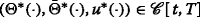is an optimal closed-loop strategy of Problem (MF-LQ) on [t,T] if and only if for any \(\xi \in L^{2}_{{\mathcal {F}}_{t}}(\Omega ;\mathbb {R}^{n}), u (·) is an optimal open-loop control of the problem with the above state equation and cost functional. This leads to the following result. ### Proposition 3.1 Let (H1)–(H2) hold. Ifis an optimal closed-loop strategy of Problem (MF-LQ) on [t,T], then $$(\Theta ^{*}(\cdot),\bar \Theta ^{*}(\cdot),0)$$ is an optimal closed-loop strategy of Problem (MF-LQ) 0 on [t,T]. ### Proof. By the preceding discussion and (Theorem 2.3, Sun 2016), we see that $$(\Theta ^{*}(\cdot),\bar \Theta ^{*}(\cdot),u^{*}(\cdot))$$ is an optimal closed-loop strategy of Problem (MF-LQ) on [t,T] if and only if for any $$\xi \in L^{2}_{{\mathcal {F}}_{t}}(\Omega ;\mathbb {R}^{n})$$, the adapted solution (X (·),Y (·),Z (·)) to the following mean-field forward-backward stochastic differential equation (MF-FBSDE, for short): \begin{aligned} \left\{\! \begin{array}{ll} dX^{*}(s)&=\left\{(A\,+\,B\Theta^{*})X^{*}\,+\,\left[\bar A\,+\,B\bar\Theta^{*}\,+\,\bar B(\Theta^{*}\,+\,\bar\Theta^{*})\right]\mathbb{E}[\!X^{*}]\! +Bu^{*}\,+\,\bar B\mathbb{E}[u^{*}]\!+b\right\}\!ds\\ &\quad+\!\left\{(C\,+\,D\Theta^{*})X^{*}\,+\,\left[\bar C\,+\,D\bar\Theta^{*}\,+\,\bar D(\Theta^{*}\,+\,\bar\Theta^{*})\right]\mathbb{E}[\!X^{*}] \!+Du^{*}\,+\,\bar D\mathbb{E}[u^{*}]\!+\sigma\!\right\}\!dW(s),\\ dY^{*}(s)&=-\left\{(A\,+\,B\Theta^{*})^{\top} Y^{*}\,+\,\left[\bar A\,+\,B\bar\Theta^{*}\,+\,\bar B(\Theta^{*}\,+\,\bar\Theta^{*})\right]^{\top}\mathbb{E}[Y^{*}]\right.\\ &\quad+(C\,+\,D\Theta^{*})^{\top} Z^{*}\,+\,\left[\bar C\,+\,D\bar\Theta^{*}\,+\,\bar D(\Theta^{*}\,+\,\bar\Theta^{*})\right]^{\top}\mathbb{E}[Z^{*}]\\ &\quad\left.+\widetilde QX^{*}\,+\,\widehat Q\mathbb{E}[\!X^{*}]\!+\widetilde S^{\top} u^{*}\,+\,\widehat S^{\top}\mathbb{E}[\!u^{*}]\!+\widetilde q\,+\,\widehat q\vphantom{(A+B\Theta^{*})^{\top} Y^{*}+\left[\bar A+B\bar\Theta^{*}+\bar B(\Theta^{*}+\bar\Theta^{*})\right]^{\top}\mathbb{E}[Y^{*}]}\right\}\!ds\,+\,Z^{*}dW(s), \qquad s\in[t,T],\\ X^{*}(t)&=\xi,\qquad Y^{*}(T)=GX^{*}(T)\,+\,\bar G\mathbb{E}[X^{*}(T)]+g+\bar g, \end{array} \right. \end{aligned} $$(3.1) satisfies$$Ru^{*}+ B^{\top} Y^{*}+ D^{\top} Z^{*}+\widetilde SX^{*}+\rho +\bar R\mathbb{E}[u^{*}]+\bar B^{\top}\mathbb{E}[Y^{*}]+\bar D^{\top}\mathbb{E}[Z^{*}]+\widehat S\mathbb{E}[X^{*}]+\bar\rho=0, $$(3.2) and the following condition holds:$$\begin{aligned} &\mathbb{E}\left\{\langle GX(T),X(T)\rangle\,+\,\left\langle\bar G\mathbb{E}[\!X(T)],\mathbb{E}[\!X(T)]\right\rangle +\!{\int_{t}^{T}} \!\left[ \langle\widetilde QX,X\rangle\,+\,2\left\langle\widetilde SX,u\right\rangle\,+\,\langle Ru,u\rangle \right] ds\right.\\ &\left.\quad+{\int_{t}^{T}} \left[\left\langle\widehat Q\mathbb{E}[X],\mathbb{E}[X]\right\rangle\,+\,2\left\langle\widehat S\mathbb{E}[\!X],\mathbb{E}[\!u]\right\rangle \,+\,\left\langle\bar R\mathbb{E}[\!u],\mathbb{E}[\!u] \right\rangle\right]\!ds\vphantom{GX(T),X(T)\rangle+\left\langle\bar G\mathbb{E}[X(T)],\mathbb{E}[X(T)]\right\rangle +{\int_{t}^{T}} \left[ \langle\widetilde QX,X\rangle+2\left\langle\widetilde SX,u\right\rangle+\langle Ru,u\rangle \right] ds}\right\} \!\geqslant0,\qquad\forall u(\cdot)\!\in\!{\mathcal{U}}[t,T], \end{aligned} $$where X(·) is the solution of$$\begin{aligned} \left\{\!\! \begin{array}{ll} dX(s)=\left\{(A+B\Theta^{\ast})X+\left[\bar A+B\bar\Theta^{\ast}+\bar B(\Theta^{\ast}+\bar\Theta^{\ast})\right]\mathbb{E}[X] +Bu+\bar B\mathbb{E}[u]\right\}ds \\ \qquad\qquad\;\; +\left\{(C+D\Theta^{\ast})X+\left[\bar C+D\bar\Theta^{\ast}+\bar D(\Theta^{\ast}+\bar\Theta^{\ast})\right]\mathbb{E}[X] +Du+\bar D\mathbb{E}[u]\right\}dW(s),\quad s\in[t,T], \\ X(t)=0. \end{array}\right. \end{aligned} $$Since the MF-FBSDE (3.1) admits a solution for each $$\xi \in L^{2}_{{\mathcal {F}}_{t}}(\Omega ;\mathbb {R}^{n})$$ and $$(\Theta ^{\ast }(\cdot),\bar \Theta ^{\ast }(\cdot),u^{\ast }(\cdot))$$ is independent of ξ, by subtracting solutions corresponding ξ and 0, the latter from the former, we see that for any $$\xi \in L^{2}_{{\mathcal {F}}_{t}}(\Omega ;\mathbb {R}^{n})$$, the following MF-FBSDE:$$\begin{aligned} \left\{\! \begin{array}{ll} dX(s)=\left\{(A\,+\,B\Theta^{\ast})X\,+\,\left[\bar A+B\bar\Theta^{\ast}\,+\,\bar B(\Theta^{\ast}\,+\,\bar\Theta^{\ast})\right]\mathbb{E}[\!X]\right\}\!ds\\ \qquad\quad~~~ +\!\left\{(C\,+\,D\Theta^{\ast})X\,+\,\left[\bar C\,+\,D\bar\Theta^{\ast}\,+\,\bar D(\Theta^{\ast}\,+\,\bar\Theta^{\ast})\right]\mathbb{E}[\!X]\!\right\}\!dW(s),\qquad s\in[t,T],\\ dY(s)=-\left\{(A\,+\,B\Theta^{\ast})^{\top} Y\,+\,\left[\bar A\,+\,B\bar\Theta^{\ast}\,+\,\bar B(\Theta^{\ast}\,+\,\bar\Theta^{\ast})\right]^{\top}\mathbb{E}[\!Y]\!+(C\,+\,D\Theta^{\ast})^{\top} Z\right.\\ \left.\qquad\qquad\quad +\!\left[\bar C\,+\,D\bar\Theta^{\ast}\,+\,\bar D(\Theta^{\ast}\,+\,\bar\Theta^{\ast})\right]^{\top}\!\mathbb{E}[\!Z]\!+\widetilde QX\,+\,\widehat Q\mathbb{E}[\!X]\right\}\!ds\,+\,ZdW(s),\quad s\in[t,T],\\ X(t)=\xi,\qquad Y(T)=GX(T)\,+\,\bar G\mathbb{E}[X(T)], \end{array} \right. \end{aligned} $$also admits an adapted solution (X(·),Y(·),Z(·)) satisfying$$B^{\top} Y+ D^{\top} Z+\widetilde SX+\bar B^{\top}\mathbb{E}[Y]+\bar D^{\top}\mathbb{E}[Z]+\widehat S\mathbb{E}[X]=0. $$It follows, again from (Theorem 2.3, Sun2016), that $$(\Theta ^{\ast }(\cdot),\bar \Theta ^{\ast }(\cdot),0)$$ is an optimal closed-loop strategy of Problem (MF-LQ)0 on [t,T]. □ Now let us look at Problem (MF-LQ)0. If we consider only closed-loop strategies of the form $$(\Theta (\cdot),\bar \Theta (\cdot),0)$$, then the state equation becomes$$\begin{aligned} \left\{ \begin{array}{ll} dX(s)=\left\{(A+B\Theta)X+\left[\bar A+\bar B\Theta+(B+\bar B)\bar\Theta\right]\mathbb{E}[X]\right\}ds\\ \qquad\quad~~~+\left\{(C+D\Theta)X+\left[\bar C+\bar D\Theta+(D+\bar D)\bar\Theta\right]\mathbb{E}[X]\right\}dW(s), \qquad s\in[t,T], \\ X(t)=\xi, \end{array}\right. \end{aligned} $$and $$\mathbb {E}[X(\cdot)]$$ satisfies$$\left\{ \begin{array}{ll} d\mathbb{E}[X(s)]=\left[A+\bar A+(B+\bar B)(\Theta+\bar\Theta)\right]\mathbb{E}[X]ds, \qquad s\in[t,T], \\ \mathbb{E}[X(t)]=\mathbb{E}[\xi]. \end{array} \right. $$By Itô’s formula, the matrices $$\mathbf {X}(s)\triangleq \mathbb {E}\left [X(s)X(s)^{\top }\right ]$$ and $$\mathbf {Y}(s)\triangleq \mathbb {E}[X(s)]\mathbb {E}[X(s)]^{\top }$$ satisfy the matrix-valued ordinary differential equations (ODEs, for short)$$ \begin{aligned} \left\{ \begin{array}{ll} \dot{\mathbf{X}}=(A+B\Theta)\mathbf{X}+\mathbf{X}(A+B\Theta)^{\top}+(C+D\Theta)\mathbf{X}(C+D\Theta)^{\top}\\ \qquad~+\left[\bar A+\bar B\Theta+(B+\bar B)\bar\Theta\right]\mathbf{Y} +\mathbf{Y}\left[\bar A+\bar B\Theta+(B+\bar B)\bar\Theta\right]^{\top}\\ \qquad~+(C+D\Theta)\mathbf{Y}\left[\bar C+\bar D\Theta+(D+\bar D)\bar\Theta\right]^{\top} +\left[\bar C+\bar D\Theta+(D+\bar D)\bar\Theta\right]\mathbf{Y}(C+D\Theta)^{\top}\\ \qquad~+\left[\bar C+\bar D\Theta+(D+\bar D)\bar\Theta\right]\mathbf{Y} \left[\bar C+\bar D\Theta+(D+\bar D)\bar\Theta\right]^{\top}, \qquad s\in[t,T],\\ \mathbf{X}(t)=\mathbb{E}[\xi\xi^{\top}], \end{array}\right. \end{aligned} $$(3.3) and$$ \begin{aligned}\left\{ \begin{array}{ll} \dot{\mathbf{Y}}=\left[A+\bar A+(B+\bar B)(\Theta+\bar\Theta)\right]\mathbf{Y} +\mathbf{Y}\left[A+\bar A+(B+\bar B)(\Theta+\bar\Theta)\right]^{\top}, \qquad s\in[t,T],\\ \mathbf{Y}(t)=\mathbb{E}[\xi]\mathbb{E}[\xi]^{\top}, \end{array}\right. \end{aligned} $$(3.4) respectively. The cost functional $$J^{0}(t,\xi ;\Theta (\cdot)X(\cdot)+\bar \Theta (\cdot)\mathbb {E}[X(\cdot)])$$ can be expressed equivalently as$$ \mathbf{J}(t,\xi;\Theta(\cdot),\bar\Theta(\cdot))=\text{tr}[G\mathbf{X}(T)+\bar G\mathbf{Y}(T)] +{\int_{t}^{T}}\text{tr}[M(s)\mathbf{X}(s)+N(s)\mathbf{Y}(s)]ds, $$(3.5) where$$\left\{\begin{array}{cll} M&=Q+\Theta^{\top} S+S^{\top}\Theta+\Theta^{\top} R\Theta, \\ N&=\bar Q+(\Theta+\bar\Theta)^{\top}\bar S+\bar S^{\top}(\Theta+\bar\Theta)+(\Theta+\bar\Theta)^{\top}\bar R(\Theta+\bar\Theta)\\ &\qquad+\bar\Theta^{\top} R\bar\Theta+\bar\Theta^{\top} S+S^{\top}\bar\Theta+\bar\Theta^{\top} R\Theta+\Theta^{\top} R\bar\Theta. \end{array} \right. $$Then we may pose the following deterministic optimal control problem. Problem (O). For any given $$(t,\xi)\in [0,T)\times L^{2}_{{\mathcal {F}}_{t}}(\Omega ;\mathbb {R}^{n})$$, find $$\Theta ^{\ast }(\cdot),\bar \Theta ^{\ast }(\cdot)\in L^{2}(t,T;\mathbb {R}^{m\times n})$$ such that$$\begin{array}{ll} \mathbf{J}(t,\xi;\Theta^{\ast}(\cdot),\bar\Theta^{\ast}(\cdot))\leqslant\mathbf{J}(t,\xi;\Theta(\cdot),\bar\Theta(\cdot)), \qquad\forall \Theta(\cdot),\bar\Theta(\cdot)\in L^{2}(t,T;\mathbb{R}^{m\times n}). \end{array} $$Rewrite (3.3)–(3.4) as$$\left\{ \begin{array}{ll} \left(\begin{array}{c}\dot{\mathbf{X}}(s)\\ \dot{\mathbf{Y}}(s)\end{array}\right) =\left(\begin{array}{c}F_{1}(\mathbf{X}(s),\mathbf{Y}(s),\Theta(s),\bar\Theta(s),s)\\ F_{2}(\mathbf{Y}(s),\Theta(s),\bar\Theta(s),s)\end{array}\right),\qquad s\in[t,T],\\ \mathbf{X}(t)=\mathbb{E}[\xi\xi^{\top}],\quad \mathbf{Y}(t)=\mathbb{E}[\xi]\mathbb{E}[\xi]^{\top}, \end{array}\right. $$and denote the integrand in (3.5) by $$L(\mathbf {X}(s),\mathbf {Y}(s),\Theta (s),\bar \Theta (s),s)$$. We present the following matrix minimum principle for Problem (O). The interested reader is referred to Athans (1968) for a proof. ### Lemma 3.2 Let (H1)–(H2) hold. Suppose that $$(\Theta ^{\ast }(\cdot),\bar \Theta ^{\ast }(\cdot))$$ is an optimal control of Problem (O) for the initial pair (t,ξ) and let (X (·),Y (·)) be the corresponding optimal state process. Then there exist matrix-valued functions P(·) and Λ(·) satisfying the following ODEs (the variable s[t,T] is suppressed)$$ \begin{aligned}\left\{\! \begin{array}{ll} \left(\begin{array}{c}\dot P\\ \dot\Lambda\end{array}\right)\,=\,-\!\left(\!\begin{array}{c} {{\partial}\over{\partial} \mathbf{X}^{\ast}}L(\mathbf{X}^{\ast},\mathbf{Y}^{\ast},\Theta^{\ast},\bar\Theta^{\ast}) \,+\,{{\partial}\over{\partial} \mathbf{X}^{\ast}}\text{tr}[\!F_{1}(\mathbf{X}^{\ast},\mathbf{Y}^{\ast},\Theta^{\ast},\bar\Theta^{\ast})P^{\top}\,+\,F_{2}(\mathbf{Y}^{\ast},\Theta^{\ast},\bar\Theta^{\ast})\Lambda^{\top}]\\ {{\partial}\over{\partial} \mathbf{Y}^{\ast}}L(\mathbf{X}^{\ast},\mathbf{Y}^{\ast},\Theta^{\ast},\bar\Theta^{\ast}) \,+\,{{\partial}\over{\partial} \mathbf{Y}^{\ast}}\text{tr}[\!F_{1}(\mathbf{X}^{\ast},\mathbf{Y}^{\ast},\Theta^{\ast},\bar\Theta^{\ast})P^{\top}\,+\,F_{2}(\mathbf{Y}^{\ast},\Theta^{\ast},\bar\Theta^{\ast})\Lambda^{\top}] \end{array}\right),\\ P(T)=G,\qquad \Lambda(T)=\bar G, \end{array}\right. \end{aligned} $$(3.6) with constraints$$ \begin{aligned} \left\{ \begin{array}{ll} {{\partial}\over{\partial} \Theta^{\ast}}L(\mathbf{X}^{\ast},\mathbf{Y}^{\ast},\Theta^{\ast},\bar\Theta^{\ast}) +{{\partial}\over{\partial} \Theta^{\ast}}\text{tr}[F_{1}(\mathbf{X}^{\ast},\mathbf{Y}^{\ast},\Theta^{\ast},\bar\Theta^{\ast})P^{\top}+F_{2}(\mathbf{Y}^{\ast},\Theta^{\ast},\bar\Theta^{\ast})\Lambda^{\top}]=0,\\ {{\partial}\over{\partial} \bar\Theta^{\ast}}L(\mathbf{X}^{\ast},\mathbf{Y}^{\ast},\Theta^{\ast},\bar\Theta^{\ast}) +{{\partial}\over{\partial} \bar\Theta^{\ast}}\text{tr}[F_{1}(\mathbf{X}^{\ast},\mathbf{Y}^{\ast},\Theta^{\ast},\bar\Theta^{\ast})P^{\top}+F_{2}(\mathbf{Y}^{\ast},\Theta^{\ast},\bar\Theta^{\ast})\Lambda^{\top}]=0. \end{array} \right. \end{aligned} $$(3.7) Now, we are ready to state and prove the principal result of this section. ### Theorem 3.3 Let (H1)–(H2) hold and t(0,T). If Problem (MF-LQ) admits an optimal closed-loop strategy on [t,T], then the GRE (2.6) is regularly solvable on [t,T]. ### Proof. Suppose thatis an optimal closed-loop strategy of Problem (MF-LQ) on [t,T]. Then, by Proposition 3.1, $$(\Theta ^{\ast }(\cdot),\bar \Theta ^{\ast }(\cdot),0)$$ is an optimal closed-loop strategy of Problem (MF-LQ) 0 on [t,T], and it follows from Definition 2.3 (i) that $$(\Theta ^{\ast }(\cdot),\bar \Theta ^{\ast }(\cdot))$$ is an optimal control of Problem (O) for any $$\xi \in L^{2}_{{\mathcal {F}}_{t}}(\Omega ;\mathbb {R}^{n})$$. Thus, by the matrix minimum principle, Lemma 3.2, there exist functions $$P(\cdot),\Lambda (\cdot):[t,T]\to \mathbb {R}^{n}$$ such that (3.6)–(3.7) hold. By a straightforward calculation, we see from the first equation in (3.6) that P(·) satisfies$$ \left\{ \begin{array}{ll} \dot P+(A+B\Theta^{\ast})^{\top} P+P(A+B\Theta^{\ast})+(C+D\Theta^{\ast})^{\top} P(C+D\Theta^{\ast})\\ \quad+\,Q+(\Theta^{\ast})^{\top} S+S^{\top}\Theta^{\ast}+(\Theta^{\ast})^{\top} R\Theta^{\ast}=0,\\ P(T)=G, \end{array} \right. $$(3.8) and from the second equation in (3.6), we see that Λ(·) satisfies$$ \begin{aligned} \left\{ \begin{array}{ll} 0=\dot\Lambda+\bar Q+(\Theta^{\ast}+\bar\Theta^{\ast})^{\top}\bar S+\bar S^{\top}(\Theta^{\ast}+\bar\Theta^{\ast}) +(\Theta^{\ast}+\bar\Theta^{\ast})^{\top}\bar R(\Theta^{\ast}+\bar\Theta^{\ast})\\ \qquad~~~+(\bar\Theta^{\ast})^{\top} R\bar\Theta^{\ast}+(\bar\Theta^{\ast})^{\top} S+S^{\top}\bar\Theta^{\ast} +(\bar\Theta^{\ast})^{\top} R\Theta^{\ast}+(\Theta^{\ast})^{\top} R\bar\Theta^{\ast}\\ \qquad~~~+\left[\bar A+\bar B\Theta^{\ast}+(B+\bar B)\bar\Theta^{\ast}\right]^{\top} P +P\left[\bar A+\bar B\Theta^{\ast}+(B+\bar B)\bar\Theta^{\ast}\right]\\ \qquad~~~+\left[C+D\Theta^{\ast}\right]^{\top} P\left[\bar C+\bar D\Theta^{\ast}+(D+\bar D)\bar\Theta^{\ast}\right]\\ \qquad~~~+\left[\bar C+\bar D\Theta^{\ast}+(D+\bar D)\bar\Theta^{\ast}\right]^{\top} P\left[C+D\Theta^{\ast}\right]\\ \qquad~~~+\left[\bar C+\bar D\Theta^{\ast}+(D+\bar D)\bar\Theta^{\ast}\right]^{\top} P \left[\bar C+\bar D\Theta^{\ast}+(D+\bar D)\bar\Theta^{\ast}\right]\\ \qquad~~~+\left[A+\bar A+(B+\bar B)(\Theta^{\ast}+\bar\Theta^{\ast})\right]^{\top}\Lambda +\Lambda\left[A+\bar A+(B+\bar B)(\Theta^{\ast}+\bar\Theta^{\ast})\right],\\ \Lambda(T)=\bar G. \end{array}\right. \end{aligned} $$(3.9) Note that P(·) and Λ(·) also solve (3.8) and (3.9), respectively. Hence, by uniqueness, we have P(·)=P(·) and Λ(·)=Λ(·). Let$$\Pi(\cdot)=P(\cdot)+\Lambda(\cdot),\qquad \Delta(\cdot)=\Theta^{\ast}(\cdot)+\bar\Theta^{\ast}(\cdot). $$Then, $$\Pi (T)=G+\bar G$$ and$$ \begin{array}{lllll} 0=\dot\Pi+Q+\bar Q+\Delta^{\top}(S+\bar S)+(S+\bar S)^{\top}\Delta+\Delta^{\top}(R+\bar R)\Delta\\ \qquad~~~+\left[A+\bar A+(B+\bar B)\Delta\right]^{\top} P+P\left[A+\bar A+(B+\bar B)\Delta\right]\\ \qquad~~~+\left[C+D\Theta^{\ast}\right]^{\top} P\left[C+\bar C+(D+\bar D)\Delta\right]\\ \qquad~~~+\left[\bar C+\bar D\Theta^{\ast}+(D+\bar D)\bar\Theta^{\ast}\right]^{\top} P\left[C+\bar C+(D+\bar D)\Delta\right]\\ \qquad~~~+\left[A+\bar A+(B+\bar B)\Delta\right]^{\top}\Lambda+\Lambda\left[A+\bar A+(B+\bar B)\Delta\right]\\ ~~=\dot\Pi+Q+\bar Q+\Delta^{\top}(S+\bar S)+(S+\bar S)^{\top}\Delta+\Delta^{\top}(R+\bar R)\Delta\\ \qquad~~~+\left[A+\bar A+(B+\bar B)\Delta\right]^{\top}\Pi+\Pi\left[A+\bar A+(B+\bar B)\Delta\right]\\ \qquad~~~+\left[C+\bar C+(D+\bar D)\Delta\right]^{\top} P\left[C+\bar C+(D+\bar D)\Delta\right]\\ ~~=\dot\Pi+\left[A+\bar A+(B+\bar B)\Delta\right]^{\top}\Pi+\Pi\left[A+\bar A+(B+\bar B)\Delta\right]\\ \qquad+Q+\bar Q+(C+\bar C)^{\top} P(C+\bar C)+\Delta^{\top}\left[R+\bar R+(D+\bar D)^{\top} P(D+\bar D)\right]\Delta\\ \qquad+\Delta^{\top}\left[(D+\bar D)^{\top} P(C+\bar C)+S+\bar S\right] +\left[(D+\bar D)^{\top} P(C+\bar C)+S+\bar S\right]^{\top}\Delta. \end{array} $$(3.10) Also, from the first equality in (3.7), we have (noting that X and Y are symmetric)$$ \begin{aligned} \begin{array}{lll} 0=2S\mathbf{X}^{\ast}+2R\Theta^{\ast}\mathbf{X}^{\ast}+2\bar S\mathbf{Y}^{\ast}+2\bar R\Theta^{\ast}\mathbf{Y}^{\ast} +2\bar R\bar\Theta^{\ast}\mathbf{Y}^{\ast}+2R\bar\Theta^{\ast}\mathbf{Y}^{\ast}\\ \qquad+\,2B^{\top} P\mathbf{X}^{\ast}+2D^{\top} PC\mathbf{X}^{\ast}+2D^{\top} PD\Theta^{\ast}\mathbf{X}^{\ast}\\ \qquad+\,2\bar B^{\top} P\mathbf{Y}^{\ast}+2\bar D^{\top} PC\mathbf{Y}^{\ast} +2D^{\top} P\left[\bar C+(D+\bar D)\bar\Theta^{\ast}\right]\mathbf{Y}^{\ast}\\ \qquad+\,2D^{\top} P\bar D\Theta^{\ast}\mathbf{Y}^{\ast}+2\bar D^{\top} PD\Theta^{\ast}\mathbf{Y}^{\ast} +2\bar D^{\top} P\left[\bar C+(D+\bar D)\bar\Theta^{\ast}\right]\mathbf{Y}^{\ast}\\ \qquad+\,2\bar D^{\top} P\bar D\Theta^{\ast}\mathbf{Y}^{\ast}+2(B+\bar B)^{\top}\Lambda \mathbf{Y}^{\ast}\\ =2\left[(R+D^{\top} PD)\Theta^{\ast}+B^{\top} P+D^{\top} PC+S\right]\mathbf{X}^{\ast}\\ \qquad+2\left\{(R+\bar R)\bar\Theta^{\ast}+(\bar R+\bar D^{\top} PD)\Theta^{\ast} +\bar B^{\top} P+\bar D^{\top} PC+\bar S\right.\\ \qquad~~~~~~~~~~\left.+(D+\bar D)^{\top} P\left[\bar C+\bar D\Theta^{\ast}+(D+\bar D)\bar\Theta^{\ast}\right] +(B+\bar B)^{\top}\Lambda \right\}\mathbf{Y}^{\ast}\\ =2\left[(R+D^{\top} PD)\Theta^{\ast}+B^{\top} P+D^{\top} PC+S\right]\mathbf{X}^{\ast}\\ \qquad+\,2\left\{-\left[(R+D^{\top} PD)\Theta^{\ast}+B^{\top} P+D^{\top} PC+S\right]\right.\\ \qquad\qquad~~\left.+(R+\bar R)\Delta+(B+\bar B)^{\top}\Pi +(D+\bar D)^{\top} P\left[C+\bar C+(D+\bar D)\Delta\right]+S+\bar S\right\}\mathbf{Y}^{\ast}\\ =2\left[(R+D^{\top} PD)\Theta^{\ast}+B^{\top} P+D^{\top} PC+S\right](\mathbf{X}^{\ast}-\mathbf{Y}^{\ast})\\ \qquad+\,2\left\{\left[R+\bar R+(D+\bar D)^{\top} P(D+\bar D)\right]\Delta+(B+\bar B)^{\top}\Pi\right.\\ \qquad\qquad\left.+(D+\bar D)^{\top} P(C+\bar C)+S+\bar S\right\}\mathbf{Y}^{\ast}. \end{array} \end{aligned} $$(3.11) Likewise, from the second equality in (3.7), we have$$ \begin{array}{ll} 2\left\{\left[R+\bar R+(D+\bar D)^{\top} P(D+\bar D)\right]\Delta+(B+\bar B)^{\top}\Pi\right.\\ \quad~\left.+(D+\bar D)^{\top} P(C+\bar C)+S+\bar S\right\}\mathbf{Y}^{\ast}=0. \end{array} $$(3.12) Let Φ(·) be the solution to the $$\mathbb {R}^{n\times n}$$-valued ODE$$\left\{\begin{array}{ll} \dot \Phi(s)=\widetilde A(s)\Phi(s),\qquad s\in[t,T],\\ \Phi(t)=I, \end{array} \right. $$where$$\widetilde A\triangleq A+\bar A+(B+\bar B)(\Theta^{\ast}+\bar\Theta^{\ast})=A+\bar A+(B+\bar B)\Delta. $$Then$$\mathbf{Y}^{\ast}(s)=\Phi(s)\mathbb{E}[\xi]\mathbb{E}[\xi]^{\top}\Phi(s)^{\top},\qquad s\in[t,T]. $$Denoting $$\bar \Sigma \equiv R+\bar R+(D+\bar D)^{\top } P(D+\bar D)$$, since (3.12) holds for all $$\xi \in L_{{\mathcal {F}}_{t}}^{2}(\Omega ;\mathbb {R}^{n})$$ and Φ(s) is invertible for all s[t,T], we must have$$ \bar\Sigma\Delta+(B+\bar B)^{\top}\Pi+(D+\bar D)^{\top} P(C+\bar C)+S+\bar S=0. $$(3.13) Now take $$\eta \in L_{{\mathcal {F}}_{t}}^{2}(\Omega ;\mathbb {R})$$ with $$\mathbb {E}\eta =0$$ and $$\mathbb {E}\eta ^{2}=1$$. Then for any $$x\in \mathbb {R}^{n}$$, the solution Y of (3.4) corresponding to $$(\Theta,\bar \Theta)=(\Theta ^{\ast },\bar \Theta ^{\ast })$$ and ξ=η x is identically zero, and the corresponding solution X of (3.3) satisfies$$\begin{aligned} \left\{\begin{array}{ll} \dot{\mathbf{X}}^{\ast}=(A+B\Theta^{\ast})\mathbf{X}^{\ast}+\mathbf{X}^{\ast}(A+B\Theta^{\ast})^{\top}+(C+D\Theta^{\ast})\mathbf{X}^{\ast}(C+D\Theta^{\ast})^{\top}, \qquad s\in[t,T],\\ \mathbf{X}^{\ast}(t)=xx^{\top}, \end{array}\right. \end{aligned} $$Let Ψ(·) be the solution to the following SDE for $$\mathbb {R}^{n\times n}$$-valued process:$$\begin{aligned} \left\{\begin{array}{ll} d\Psi(s)=\left[A(s)+B(s)\Theta^{\ast}(s)\right]\Psi(s)ds \,+\,\left[C(s)+D(s)\Theta^{\ast}(s)\right]\Psi(s)dW(s),\qquad s\in[t,T],\\ \Psi(t)=I. \end{array}\right. \end{aligned} $$Then we have$$\mathbf{X}^{\ast}(s)=\mathbb{E}\left[\Psi(s)xx^{\top}\Psi(s)^{\top}\right],\qquad s\in[t,T]. $$Hence, denoting ΣR+D PD, we obtain from (3.11) that$$\left(\Sigma\Theta^{\ast}+B^{\top} P+D^{\top} PC+S\right)\mathbb{E}\left[\Psi xx^{\top}\Psi^{\top}\right]=0, \qquad\forall x\in\mathbb{R}^{n}, $$which implies Σ Θ +B P+D PC+S=0. It follows that $${\mathcal {R}}(B^{\top } P+D^{\top } PC+S)\subseteq {\mathcal {R}}(\Sigma)$$. Moreover, since Σ Σ is an orthogonal projection, we have$$\Sigma^{\dag}(B^{\top} P+D^{\top} PC+S)\in L^{2}(t,T;\mathbb{R}^{m\times n}), $$and$$ \Theta^{\ast}=-\Sigma^{\dag}(B^{\top} P+D^{\top} PC+S)+(I-\Sigma^{\dag}\Sigma)\theta, $$(3.14) for some $$\theta (\cdot)\in L^{2}(t,T;\mathbb {R}^{m\times n})$$. Similarly, from (3.13) we have$${\mathcal{R}}\left((B+\bar B)^{\top}\Pi+(D+\bar D)^{\top} P(C+\bar C)+(S+\bar S)\right) \subseteq{\mathcal{R}}(\bar \Sigma), \bar\Sigma^{\dag}\left[(B+\bar B)^{\top}\Pi+(D+\bar D)^{\top} P(C+\bar C)+(S+\bar S)\right] \in L^{2}(t,T;\mathbb{R}^{m\times n}), $$and$$ \Delta=-\bar\Sigma^{\dag}\left[(B+\bar B)^{\top}\Pi+(D+\bar D)^{\top} P(C+\bar C)+(S+\bar S)\right] +\left(I-\bar\Sigma^{\dag}\bar\Sigma\right)\tau, $$(3.15) for some $$\tau (\cdot)\in L^{2}(t,T;\mathbb {R}^{m\times n})$$. Substituting (3.14) and (4.8) back into (3.8) and (3.10), respectively, we see that (P(·),Π(·)) satisfies the GRE (2.6). In order to show that (P(·),Π(·)) is regular, it remains to prove that$$\Sigma\equiv R+D^{\top} PD\geqslant0,\qquad\bar\Sigma\equiv R+\bar R+(D+\bar D)^{\top} P(D+\bar D)\geqslant0. $$For this we take any $$u(\cdot)\in {\mathcal {U}}[t,T]$$ and let X(·) be the solution to$$ \begin{aligned} \left\{\begin{array}{ll} dX(s)=\left\{A(s)X(s)\,+\,\bar A(s)\mathbb{E}[X(s)]\!+B(s)u(s)\,+\,\bar B(s)\mathbb{E}[u(s)]\right\}ds\\ \qquad\qquad~ +\!\left\{C(s)X(s)\,+\,\bar C(s)\mathbb{E}[X(s)]\!+D(s)u(s)\,+\,\bar D(s)\mathbb{E}[u(s)]\right\}dW(s),\qquad s\in[t,T],\\ X(t)=0. \end{array}\right. \end{aligned} $$(3.16) Applying Itô’s formula to $$s\mapsto \langle P(s)(X(s)-\mathbb {E}[X(s)]),X(s)-\mathbb {E}[X(s)]\rangle$$ and $$s\mapsto \langle \Pi (s)\mathbb {E}[X(s)],\mathbb {E}[X(s)]\rangle$$, we have$$ \begin{aligned} J^{0}(t,0;u(\cdot))\\ &=\mathbb{E}\left\{\vphantom{+{\int_{t}^{T}}\left\langle\left(\begin{array}{cc}Q+\bar Q&(S+\bar S)^{\top}\\S+\bar S&R+\bar R\end{array}\right) \left(\begin{array}{c}\mathbb{E}[X]\\ \mathbb{E}[u]\end{array}\right), \left(\begin{array}{c}\mathbb{E}[X]\\ \mathbb{E}[u]\end{array}\right)\right\rangle ds}\langle G(X(T)\,-\,\mathbb{E}[\!X(T)]),X(T)\,-\,\mathbb{E}[X(T)]\rangle\,+\,\left\langle(G+\!\bar G)\mathbb{E}[X(T)],\mathbb{E}[X(T)]\right\rangle\right.\\ &\qquad~~+{\int_{t}^{T}}\left\langle\left(\begin{array}{ll}Q&S^{\top} \\ S&R\end{array}\right) \left(\begin{array}{c}X-\mathbb{E}[X]\\ u-\mathbb{E}[u]\end{array}\right), \left(\begin{array}{c}X-\mathbb{E}[X]\\ u-\mathbb{E}[u]\end{array}\right)\right\rangle ds\\ &\qquad~~\left.+{\int_{t}^{T}}\left\langle\left(\begin{array}{cc}Q+\bar Q&(S+\bar S)^{\top}\\S+\bar S&R+\bar R\end{array}\right) \left(\begin{array}{c}\mathbb{E}[X]\\ \mathbb{E}[u]\end{array}\right), \left(\begin{array}{c}\mathbb{E}[X]\\ \mathbb{E}[u]\end{array}\right)\right\rangle ds\right\}\\ &=\! \mathbb{E}\!{\int_{t}^{T}}\!\left\{\!\!\vphantom{+{\int_{t}^{T}}\left\langle\left(\begin{array}{cc}Q+\bar Q&(S+\bar S)^{\top}\\S+\bar S&R+\bar R\end{array}\right) \left(\begin{array}{c}\mathbb{E}[X]\\ \mathbb{E}[u]\end{array}\right), \left(\begin{array}{c}\mathbb{E}[X]\\ \mathbb{E}[u]\end{array}\right)\right\rangle ds}\left\langle\dot P(X\,-\,\mathbb{E}[X]),X\,-\,\mathbb{E}[\!X]\right\rangle +\!\left\langle P\left\{A(X\,-\,\mathbb{E}[\!X])\,+\,B(u\,-\,\mathbb{E}[u])\right\},X\,-\,\mathbb{E}[\!X]\right\rangle\right.\\ &\qquad\qquad~+\left\langle P(X\,-\,\mathbb{E}[X]),A(X\,-\,\mathbb{E}[X])\,+\,B(u\,-\,\mathbb{E}[\!u])\right\rangle\\ &\qquad\qquad~ +\langle P\left\{C(X\,-\,\mathbb{E}[X])\,+\,D(u\,-\,\mathbb{E}[u])\,+\,(C\,+\,\bar C)\mathbb{E}[X]\!+(D\,+\,\bar D)\mathbb{E}[u]\right\},\\ &\left.\qquad\qquad\qquad C(X\,-\,\mathbb{E}[X])\,+\,D(u\,-\,\mathbb{E}[u])\,+\,(C\,+\,\bar C)\mathbb{E}[X]\!+(D\,+\,\bar D)\mathbb{E}[u]\rangle\vphantom{+{\int_{t}^{T}}\left\langle\left(\begin{array}{cc}Q+\bar Q&(S+\bar S)^{\top}\\S+\bar S&R+\bar R\end{array}\right) \left(\begin{array}{c}\mathbb{E}[X]\\ \mathbb{E}[u]\end{array}\right), \left(\begin{array}{c}\mathbb{E}[X]\\ \mathbb{E}[u]\end{array}\right)\right\rangle ds}\right\}ds\\ &\quad +\,{\int_{t}^{T}}\left\{\left\langle\dot\Pi\mathbb{E}[X],\mathbb{E}[X]\right\rangle +\left\langle\Pi\left\{(A+\bar A)\mathbb{E}[X]+(B+\bar B)\mathbb{E}[u]\right\},\mathbb{E}[X]\right\rangle\right.\\ &\qquad\qquad~+\left.\left\langle\Pi\mathbb{E}[X],(A+\bar A)\mathbb{E}[X]+(B+\bar B)\mathbb{E}[u]\right\rangle\right\}ds\\ &\quad +\,\mathbb{E}{\int_{t}^{T}}\left\{\langle Q(X-\mathbb{E}[X]),X-\mathbb{E}[X]\rangle +2\langle S(X-\mathbb{E}[X]),u-\mathbb{E}[u]\rangle \right. \\ &\left.\qquad\qquad~+\langle R(u-\mathbb{E}[u]),u-\mathbb{E}[u]\rangle\right\}ds\\ &\quad+\!{\int_{t}^{T}}\!\!\left\{\left\langle(Q\,+\,\bar Q)\mathbb{E}[\!X],\mathbb{E}[\!X]\right\rangle\,+\,2\left\langle(S\,+\,\bar S)\mathbb{E}[\!X],\mathbb{E}[u]\right\rangle \,+\,\left\langle(R\,+\,\bar R)\mathbb{E}[u],\mathbb{E}[u]\right\rangle\right\}ds\\ &=\mathbb{E}{\int_{t}^{T}}\left\{\left\langle\left(\dot P+PA+A^{\top} P+C^{\top} PC+Q\right)(X-\mathbb{E}[X]),X-\mathbb{E}[X]\right\rangle\right.\\ &\qquad\qquad~ +2\left\langle\left(B^{\top} P+D^{\top} PC+S\right)(X-\mathbb{E}[X]),u-\mathbb{E}[u]\right\rangle \\ &\qquad\qquad~+\left.\left\langle\left(R+D^{\top} PD\right)(u-\mathbb{E}[u]),u-\mathbb{E}[u]\right\rangle\right\}ds\\ &+\,{\int_{t}^{T}}\!\!\left\{\left\langle\left[\dot\Pi\,+\,\Pi(A\,+\,\bar A)\,+\,(A\,+\,\bar A)^{\top}\Pi\,+\,(C\,+\,\bar C)^{\top} P(C\,+\,\bar C) \,+\,Q\,+\,\bar Q\right]\mathbb{E}[\!X],\mathbb{E}[\!X]\right\rangle\right.\\ \qquad\qquad~ &+2\left\langle\left[(B+\bar B)^{\top}\Pi+(D+\bar D)^{\top} P(C+\bar C)+S+\bar S\,\right]\mathbb{E}[X],\mathbb{E}[u]\right\rangle\\ &\left.\qquad\qquad~ +\left\langle\left[R+\bar R+(D+\bar D)^{\top} P(D+\bar D)\right]\mathbb{E}[u],\mathbb{E}[u]\right\rangle\right\}ds\\ &=\mathbb{E}{\int_{t}^{T}}\left\{\left\langle(\Theta^{\ast})^{\top}\Sigma\Theta^{\ast}(X\,-\,\mathbb{E}[X]),X\,-\,\mathbb{E}[X]\right\rangle \,-\,2\langle\Sigma\Theta^{\ast}(X-\mathbb{E}[X]),u-\mathbb{E}[u]\rangle \right. \\ &\left.\qquad\qquad~+\langle\Sigma(u-\mathbb{E}[u]),u-\mathbb{E}[u]\rangle{\vphantom{1^{1^{1}}}}\right\}ds\\ \quad &+\,{\int_{t}^{T}}\left\{\left\langle\Delta^{\top}\bar\Sigma\Delta\mathbb{E}[X],\mathbb{E}[X]\right\rangle-2\left\langle\bar\Sigma\Delta\mathbb{E}[X],\mathbb{E}[u]\right\rangle +\left\langle\bar\Sigma\mathbb{E}[u],\mathbb{E}[u]\right\rangle\right\}ds\\ &=\mathbb{E}{\int_{t}^{T}}\left\langle\Sigma\left\{u-\mathbb{E}[u]-\Theta^{\ast}(X-\mathbb{E}[X])\right\},u-\mathbb{E}[u]-\Theta^{\ast}(X-\mathbb{E}[X])\right\rangle ds\\ &\quad+\,{\int_{t}^{T}}\left\langle\bar\Sigma\left(\mathbb{E}[u]-\Delta\mathbb{E}[X]\right),\mathbb{E}[u]-\Delta\mathbb{E}[X]\right\rangle ds. \end{aligned} $$Since $$(\Theta ^{\ast }(\cdot),\bar \Theta ^{\ast }(\cdot),0)$$ is an optimal closed-loop strategy of Problem (MF-LQ)0 on [t,T], we have$$ \begin{array}{ll} \mathbb{E}{\int_{t}^{T}}\left\langle\Sigma\left\{u-\mathbb{E}[u]-\Theta^{\ast}(X-\mathbb{E}[X])\right\},u-\mathbb{E}[u]-\Theta^{\ast}(X-\mathbb{E}[X])\right\rangle ds\\ \quad~+{\int_{t}^{T}}\left\langle\bar\Sigma\left(\mathbb{E}[u]-\Delta\mathbb{E}[X]\right),\mathbb{E}[u]-\Delta\mathbb{E}[X]\right\rangle ds\\ =J^{0}(t,0;u(\cdot))\geqslant J^{0}(t,0;\Theta^{\ast}(\cdot)X^{\ast}(\cdot)+\bar\Theta^{\ast}(\cdot)\mathbb{E}[X^{\ast}(\cdot)])=0,\!\! \qquad\forall u(\cdot)\in{\mathcal{U}}[t,T]. \end{array} $$(3.17) Note that for any $$u(\cdot)\in {\mathcal {U}}[t,T]$$ of the form$$u(s)=\Theta^{\ast}(s)X(s)+v(s)W(s),\qquad v(\cdot)\in L^{2}(t,T;\mathbb{R}^{m}), $$the corresponding solution X(·) of (3.16) satisfies $$\mathbb {E}[X(\cdot)]=0$$ and hence $$\mathbb {E}[u(\cdot)]=0$$. Then (3.17) yields$$\begin{array}{lll} 0&\leqslant\mathbb{E}{\int_{t}^{T}}\left\langle\Sigma(s)[u(s)-\Theta^{\ast}(s)X(s)],u(s)-\Theta^{\ast}(s)X(s)\right\rangle ds\\ &=\mathbb{E}{\int_{t}^{T}}\langle\Sigma(s)v(s)W(s),v(s)W(s)\rangle ds\\ &\leqslant T{\int_{t}^{T}}\langle\Sigma(s)v(s),v(s)\rangle ds, \qquad\forall v(\cdot)\in L^{2}(t,T;\mathbb{R}^{m}), \end{array} $$which implies that $$\Sigma \geqslant 0$$. Likewise, for any $$u(\cdot)\in {\mathcal {U}}[t,T]$$ of the form$$u(s)=\Theta^{\ast}(s)\left\{X(s)-\mathbb{E}[X(s)]\right\}+\Delta(s)\mathbb{E}[X(s)]+v(s),\qquad v(\cdot)\in L^{2}(t,T;\mathbb{R}^{m}), $$the corresponding solution X(·) of (3.16) satisfies$$u(s)-\mathbb{E}[u(s)]=\Theta^{\ast}(s)\left\{X(s)-\mathbb{E}[X(s)]\right\},\qquad \mathbb{E}[u(s)]-\Delta(s)\mathbb{E}[X(s)]=v(s). $$Then (3.17) yields$$\begin{array}{lll} 0&\leqslant&{\int_{t}^{T}}\left\langle\bar\Sigma(s)\left\{\mathbb{E}[u(s)]-\Delta(s)\mathbb{E}[X(s)]\right\},\mathbb{E}[u(s)]-\Delta(s)\mathbb{E}[X(s)]\right\rangle ds\\ &=&{\int_{t}^{T}}\left\langle\bar\Sigma(s)v(s),v(s)\right\rangle ds, \qquad \forall v(\cdot)\in L^{2}(t,T;\mathbb{R}^{m}), \end{array} $$which implies that $$\bar \Sigma \geqslant 0$$. The proof is completed. □ ## Characterization of Closed-Loop Solvability The aim of this section is to provide a characterization of the closed-loop solvability of Problem (MF-LQ) in terms of the GRE (2.6), a linear backward stochastic differential equation (BSDE, for short), and a linear terminal value problem of ODE. In the case of Problem (MF-LQ)0, it turns out that the regular solvability of the GRE (2.6) is not only necessary but also sufficient for the existence of an optimal closed-loop strategy. ### Theorem 4.1 Let (H1)–(H2) hold and t(0,T). Then Problem (MF-LQ) is closed-loop solvable on [t,T] if and only if the following hold: (i) The GRE (2.6) admits a regular solution $$(P(\cdot),\Pi (\cdot))\in C([t,T];\mathbb {S}^{n})\times C([t,T];\mathbb {S}^{n})$$. (ii) The adapted solution (η(·),ζ(·)) to the BSDE$$ \left\{ \begin{array}{ll} d\eta(s)=-\left[(A+B\Theta)^{\top}\eta+(C+D\Theta)^{\top}\zeta+(C+D\Theta)^{\top} P\sigma\right.\\ \left.\qquad\qquad~~~+\Theta^{\top}\rho+Pb+q\right]ds+\zeta dW(s),\qquad s\in[t,T],\\ \eta(T)=g, \end{array}\right. $$(4.1) satisfies$$ \begin{aligned} \left\{\!\! \begin{array}{ll} B^{\top}(\eta\,-\,\mathbb{E}[\!\eta])\,+\,D^{\top}(\zeta\,-\,\mathbb{E}[\zeta])\,+\,D^{\top} P(\sigma\,-\,\mathbb{E}[\!\sigma])\,+\,\rho\,-\,\mathbb{E}[\rho]\!\in\!{\mathcal{R}}(\Sigma), \qquad\mathrm{a.e.}~s\!\in[t,T],~\mathrm{a.s.}\\ \varphi\equiv\,-\,\Sigma^{\dag}\!\left\{B^{\top}(\eta\,-\,\mathbb{E}[\!\eta])\,+\,D^{\top}(\zeta\,-\,\mathbb{E}[\zeta])\,+\,D^{\top} P(\sigma\,-\,\mathbb{E}[\sigma]) \,+\,\rho\,-\,\mathbb{E}[\rho]\right\}\!\in\! L_{\mathbb{F}}^{2}(t,T;\mathbb{R}^{m}), \end{array}\right. \end{aligned} $$(4.2) and the solution $$\bar \eta (\cdot)$$ to the ODE$$ \left\{ \begin{array}{ll} \dot{\bar\eta}+\left[(A+\bar A)+(B+\bar B)\Gamma\right]^{\top}\bar\eta +\Gamma^{\top}\left\{(D+\bar D)^{\top}\left(P\mathbb{E}[\sigma]+\mathbb{E}[\zeta]\right)+\mathbb{E}[\rho]+\bar\rho\right\}\\ ~~+(C+\bar C)^{\top}\left(P\mathbb{E}[\sigma]+\mathbb{E}[\zeta]\right)+\mathbb{E}[q]+\bar q+\Pi\mathbb{E}[b]=0,\qquad\mathrm{a.e.}~s\in[t,T],\\ \bar\eta(T)=\mathbb{E}[g]+\bar g, \end{array}\right. $$(4.3) satisfies$$ \begin{aligned} \left\{ \begin{array}{ll} (B+\bar B)^{\top}\bar\eta+(D+\bar D)^{\top}(P\mathbb{E}[\sigma]+\mathbb{E}[\zeta]) +\mathbb{E}[\rho]+\bar\rho\in{\mathcal{R}}(\bar\Sigma),\qquad\mathrm{a.e.}~s\in[t,T],\\ \bar\varphi\equiv-\bar\Sigma^{\dag}\left\{(B+\bar B)^{\top}\bar\eta +(D+\bar D)^{\top}\left(P\mathbb{E}[\sigma]+\mathbb{E}[\zeta]\right)+\mathbb{E}[\rho]+\bar\rho\right\}\in L^{2}(t,T;\mathbb{R}^{m}), \end{array}\right. \end{aligned} $$(4.4) where$$ \left\{ \begin{array}{lll} \Sigma&=&R+D^{\top} PD,\\ \bar\Sigma&=&R+\bar R+(D+\bar D)^{\top} P(D+\bar D),\\ \Theta&=&-\,\Sigma^{\dag}(B^{\top} P+D^{\top} PC+S),\\ \Gamma&=&-\,\bar\Sigma^{\dag}\left[(B+\bar B)^{\top}\Pi+(D+\bar D)^{\top} P(C+\bar C)+(S+\bar S)\right]. \end{array} \right. $$(4.5) In the above case, the optimal closed-loop strategy $$(\Theta ^{\ast }(\cdot),\bar \Theta ^{\ast }(\cdot),u^{\ast }(\cdot))$$ admits the following representation:$$ \left\{ \begin{array}{lll} \Theta^{\ast}&=&\Theta+(I-\Sigma^{\dag}\Sigma)\theta,\\ \bar\Theta^{\ast}&=&\Gamma-\Theta+(I-\bar\Sigma^{\dag}\bar\Sigma)\tau-(I-\Sigma^{\dag}\Sigma)\theta,\\ u^{\ast}&=&\varphi+\bar\varphi+(I-\Sigma^{\dag}\Sigma)(\nu-\mathbb{E}[\nu])+(I-\bar\Sigma^{\dag}\bar\Sigma)\bar\nu, \end{array}\right. $$(4.6) where $$\theta (\cdot),\tau (\cdot)\in L^{2}(t,T;\mathbb {R}^{m\times n})$$, $$\nu (\cdot)\in L_{\mathbb {F}}^{2}(t,T;\mathbb {R}^{m})$$, and $$\bar \nu (\cdot)\in L^{2}(t,T;\mathbb {R}^{m})$$. Moreover, the value V(t,ξ) is given by$${} \begin{aligned} \begin{array}{ll} V(t,\xi)=\mathbb{E}\langle P(t)(\xi-\mathbb{E}[\xi])+2\eta(t),\xi-\mathbb{E}[\xi]\rangle\! +\!\langle\Pi(t)\mathbb{E}[\xi]+2\bar\eta(t),\mathbb{E}[\xi]\rangle\\ \qquad\quad\ ~~ +\mathbb{E}{\int_{t}^{T}}\left\{\langle P\sigma,\sigma\rangle\,+\,2\langle\eta,b\,-\,\mathbb{E}[b]\rangle \,+\,2\langle\zeta,\sigma\rangle\,+\,2\langle\bar\eta,\mathbb{E}[b]\rangle \,-\,\langle\Sigma\varphi,\varphi\rangle-\left\langle\bar\Sigma\bar\varphi,\bar\varphi\right\rangle\right\}ds. \end{array} \end{aligned} $$(4.7) ### Proof. Necessity. Suppose that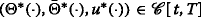is an optimal closed-loop strategy of Problem (MF-LQ) on [t,T]. Then it follows from Theorem 3.3 that the GRE (2.6) admits a regular solution (P(·),Π(·)). To determine u (·), let (X (·),Y (·),Z (·)) be the adapted solution of (3.1). Proceeding as in the proof of Proposition 3.1, we see that (X (·),Y (·),Z (·)) satisfies (3.2). Now, let$$ \Delta(\cdot)=\Theta^{\ast}(\cdot)+\bar \Theta^{\ast}(\cdot), $$(4.8) and define$$ \left\{\begin{array}{lll} \alpha&=&(Y^{\ast}-\mathbb{E}[Y^{\ast}])-P(X^{\ast}-\mathbb{E}[X^{\ast}]),\\ \beta&=&Z^{\ast}-P(C+D\Theta^{\ast})(X^{\ast}-\mathbb{E}[X^{\ast}])-PD(u^{\ast}-\mathbb{E}[u^{\ast}])-P\sigma\\ &~&-\,P\left[C+\bar C+(D+\bar D)\Delta\right]\mathbb{E}[X^{\ast}]-P(D+\bar D)\mathbb{E}[u^{\ast}],\\ \widetilde\eta&=&\mathbb{E}[Y^{\ast}]-\Pi\mathbb{E}[X^{\ast}]. \end{array} \right. $$(4.9) By the terminal value of Y (T) (see (3.1)) and P(T)=G, we obtain$$ \alpha(T)=g-\mathbb{E}[g]. $$(4.10) Also, making use of $$\Pi (T)=G+\bar G$$, we obtain$$ \widetilde\eta(T)=\mathbb{E}[g]+\bar g. $$(4.11) Further, it is obvious that$$ \mathbb{E}[\beta]=\mathbb{E}[Z^{\ast}]-P\mathbb{E}[\sigma]-P\left[C+\bar C+(D+\bar D)\Delta\right]\mathbb{E}[X^{\ast}]-P(D+\bar D)\mathbb{E}[u^{\ast}], $$(4.12) and$$ \begin{aligned} \beta-\mathbb{E}[\beta]=Z^{\ast}-\mathbb{E}[Z^{\ast}]-P(C+D\Theta^{\ast})\left(X^{\ast}-\mathbb{E}[X^{\ast}]\right)-PD\left(u^{\ast}-\mathbb{E}[u^{\ast}]\right)-P(\sigma-\mathbb{E}[\sigma]). \end{aligned} $$(4.13) We now want to show that$$ \alpha(\cdot)=\eta(\cdot)-\mathbb{E}[\eta(\cdot)],\qquad\beta(\cdot)=\zeta(\cdot),\qquad\widetilde\eta(\cdot)=\bar\eta(\cdot). $$(4.14) To this end, we recall from the proof of Theorem 3.3 that$$ \left\{ \begin{array}{ll} \dot P+(A+B\Theta^{\ast})^{\top} P+P(A+B\Theta^{\ast})+(C+D\Theta^{\ast})^{\top} P(C+D\Theta^{\ast})\\ \quad+\,Q+(\Theta^{\ast})^{\top} S+S^{\top}\Theta^{\ast}+(\Theta^{\ast})^{\top} R\Theta^{\ast}=0,\\ \Sigma\Theta^{\ast}+B^{\top} P+D^{\top} PC+S=0,\\ \Theta^{\ast}=\Theta+(I-\Sigma^{\dag}\Sigma)\theta \quad\text{for some }\theta(\cdot)\in L^{2}(t,T;\mathbb{R}^{m\times n}), \end{array}\right. $$(4.15) and (recall (4.5) and (4.8))$$ \begin{aligned} \left\{ \begin{array}{ll} \dot\Pi+\left[A+\bar A+(B+\bar B)\Delta\right]^{\top}\Pi+\Pi\left[A+\bar A+(B+\bar B)\Delta\right]\\ \quad+\,Q+\bar Q+(C+\bar C)^{\top} P(C+\bar C)+\Delta^{\top}\left[R+\bar R+(D+\bar D)^{\top} P(D+\bar D)\right]\Delta\\ \quad+\,\Delta^{\top}\left[(D+\bar D)^{\top} P(C+\bar C)+S+\bar S\right] +\left[(D+\bar D)^{\top} P(C+\bar C)+S+\bar S\right]^{\top}\Delta=0,\\ \bar\Sigma\Delta+(B+\bar B)^{\top}\Pi+(D+\bar D)^{\top} P(C+\bar C)+S+\bar S=0,\\ \Delta=\Gamma+\left(I-\bar\Sigma^{\dag}\bar\Sigma\right)\tau \quad\text{for some}~\tau(\cdot)\in L^{2}(t,T;\mathbb{R}^{m\times n}). \end{array} \right. \end{aligned} $$(4.16) Then we have$${} \begin{aligned} \begin{array}{lll} d\alpha&=d(Y^{\ast}-\mathbb{E}[Y^{\ast}])-\dot P(X^{\ast}-\mathbb{E}[X^{\ast}])ds-Pd(X^{\ast}-\mathbb{E}[X^{\ast}])\\ &=-\left\{(A+B\Theta^{\ast})^{\top}(Y^{\ast}-\mathbb{E}[Y^{\ast}])+(C+D\Theta^{\ast})^{\top}(Z^{\ast}-\mathbb{E}[Z^{\ast}])\right.\\ &\left.\quad~~+\widetilde Q(X^{\ast}-\mathbb{E}[X^{\ast}])+\widetilde S^{\top}(u^{\ast}-\mathbb{E}[u^{\ast}])+\widetilde q-\mathbb{E}[\widetilde q\,]\right\}ds+Z^{\ast}dW\\ &\quad-\,\dot P(X^{\ast}\,-\,\mathbb{E}[X^{\ast}])ds\,-\,P\left\{(A\,+\,B\Theta^{\ast})(X^{\ast}\,-\,\mathbb{E}[X^{\ast}])+B(u^{\ast}-\mathbb{E}[u^{\ast}])+b-\mathbb{E}[b]\right\}ds\\ &\quad-\,P\left\{(C+D\Theta^{\ast})(X^{\ast}-\mathbb{E}[X^{\ast}])+\left[C+\bar C+(D+\bar D)\Delta\right]\mathbb{E}[X^{\ast}]\right.\\ &\left.\qquad~+D(u^{\ast}-\mathbb{E}[u^{\ast}])+(D+\bar D)\mathbb{E}[u^{\ast}]+\sigma\right\}dW\\ &=-\left\{(A+B\Theta^{\ast})^{\top}\alpha+(A+B\Theta^{\ast})^{\top} P(X^{\ast}-\mathbb{E}[X^{\ast}])\right.\\ &\quad~~+(\!C\,+\,D\Theta^{\ast})^{\top}\!\left[\beta\,-\,\mathbb{E}[\!\beta] \,+\,P(C\,+\,D\Theta^{\ast})(X^{\ast}\,-\,\mathbb{E}[\!X^{\ast}]\!)\,+\,PD(u^{\ast}\,-\,\mathbb{E}[\!u^{\ast}])\,+\,P(\sigma\,-\,\mathbb{E}[\!\sigma])\right]\\ &\quad~~+\widetilde Q(X^{\ast}\,-\,\mathbb{E}[X^{\ast}])\,+\,\left[S^{\top}\,+\,(\Theta^{\ast})^{\top} R\right](u^{\ast}\,-\,\mathbb{E}[u^{\ast}]) \,+\,q\,-\,\mathbb{E}[q]+(\Theta^{\ast})^{\top}(\rho\,-\,\mathbb{E}[\rho])\\ &\left.\quad~+\!\dot P(X^{\ast}\!\,-\,\!\mathbb{E}[\!X^{\ast}]\!)\,+\,P(A\!\,+\,\!B\Theta^{\ast})(X^{\ast}\!\,-\,\!\mathbb{E}[\!X^{\ast}]) \,+\,PB(u^{\ast}\!\,-\,\mathbb{E}[\!u^{\ast}])\,+\,P(b\,-\,\mathbb{E}[\!b])\right\}\!ds\,+\,\beta dW\\ &=-\left\{(A+B\Theta^{\ast})^{\top}\alpha+(C+D\Theta^{\ast})^{\top}(\beta-\mathbb{E}[\beta])+(C+D\Theta^{\ast})^{\top} P(\sigma-\mathbb{E}[\sigma])\right.\\ &\left.\quad~~+(\Theta^{\ast})^{\top}(\rho-\mathbb{E}[\rho])+P(b-\mathbb{E}[b])+q-\mathbb{E}[q]\right\}ds+\beta dW\\ &\quad-\,\left\{\left[\dot P\,+\,(A\,+\,B\Theta^{\ast})^{\top} P\,+\,P(A\,+\,B\Theta^{\ast}) \,+\,(C\,+\,D\Theta^{\ast})^{\top} P(C\,+\,D\Theta^{\ast})+\widetilde Q\,\right](X^{\ast}-\mathbb{E}[X^{\ast}])\right.\\ &\left.\quad~~+\left[(C+D\Theta^{\ast})^{\top} PD+S^{\top}+(\Theta^{\ast})^{\top} R+PB\right](u^{\ast}-\mathbb{E}[u^{\ast}])\right\}ds\\ &=-\,\left\{(A+B\Theta^{\ast})^{\top}\alpha+(C+D\Theta^{\ast})^{\top}(\beta-\mathbb{E}[\beta])+(C+D\Theta^{\ast})^{\top} P(\sigma-\mathbb{E}[\sigma])\right.\\ &\left.\quad~~+(\Theta^{\ast})^{\top}(\rho-\mathbb{E}[\rho])+P(b-\mathbb{E}[b])+q-\mathbb{E}[q]\right\}ds+\beta dW. \end{array} \end{aligned} $$Also, we have$$\begin{aligned} \begin{array}{lll} -{d\widetilde\eta\over ds}&=-\,{d\mathbb{E}[Y^{\ast}]\over ds}+\dot\Pi\mathbb{E}[X^{\ast}]+\Pi{d\mathbb{E}[X^{\ast}]\over ds}\\ &=\left[A+\bar A+(B+\bar B)\Delta\right]^{\top}\mathbb{E}[Y^{\ast}]+\left[C+\bar C+(D+\bar D)\Delta\right]^{\top}\mathbb{E}[Z^{\ast}]\\ &\quad~~+\,\left(\widetilde Q+\widehat Q\right)\mathbb{E}[X^{\ast}]+\left(\widetilde S+\widehat S\,\right)^{\top}\mathbb{E}[u^{\ast}] +\mathbb{E}[\widetilde q\,]+\widehat q+\dot\Pi\mathbb{E}[X^{\ast}]\\ &\quad~~+\,\Pi\left[A+\bar A+(B+\bar B)\Delta\right]\mathbb{E}[X^{\ast}]+\Pi(B+\bar B)\mathbb{E}[u^{\ast}]+\Pi\mathbb{E}[b]\\ &=\left[A+\bar A+(B+\bar B)\Delta\right]^{\top}\widetilde\eta+\left[A+\bar A+(B+\bar B)\Delta\right]^{\top}\Pi\mathbb{E}[X^{\ast}]\\ &\quad~~+\,\left[C+\bar C+(D+\bar D)\Delta\right]^{\top}\left\{\mathbb{E}[\beta]+P\mathbb{E}[\sigma] +P\left[C+\bar C+(D+\bar D)\Delta\right]\mathbb{E}[X^{\ast}]\right.\\ &\left.\qquad~~+P(D+\bar D)\mathbb{E}[u^{\ast}]\right\}\\ &\quad~~+\,\left(\widetilde Q+\widehat Q\right)\mathbb{E}[X^{\ast}]+\left(\widetilde S+\widehat S\,\right)^{\top}\mathbb{E}[u^{\ast}] +\mathbb{E}[q]+\bar q+\Delta^{\top}(\mathbb{E}[\rho]+\bar\rho)+\dot\Pi\mathbb{E}[X^{\ast}]\\ &\quad~~+\,\Pi\left[A+\bar A+(B+\bar B)\Delta\right]\mathbb{E}[X^{\ast}]+\Pi(B+\bar B)\mathbb{E}[u^{\ast}]+\Pi\mathbb{E}[b]\\ &=\left[A+\bar A+(B+\bar B)\Delta\right]^{\top}\widetilde\eta +\Delta^{\top}\left\{(D+\bar D)^{\top}(P\mathbb{E}[\sigma]+\mathbb{E}[\beta])+\mathbb{E}[\rho]+\bar\rho\right\}\\ &\quad~~+\,(C+\bar C)^{\top}(P\mathbb{E}[\sigma]+\mathbb{E}[\beta])+\mathbb{E}[q]+\bar q+\Pi\mathbb{E}[b]\\ &\quad~~+\,\left\{\dot\Pi+\left[A+\bar A+(B+\bar B)\Delta\right]^{\top}\Pi+\Pi\left[A+\bar A+(B+\bar B)\Delta\right]\right.\\ &\left.\quad~~+\left[C+\bar C+(D+\bar D)\Delta\right]^{\top} P\left[C+\bar C+(D+\bar D)\Delta\right]+\widetilde Q+\widehat Q\right\}\mathbb{E}[X^{\ast}]\\ &\quad~~+\,\left\{\Pi(B+\bar B)+\left[C+\bar C+(D+\bar D)\Delta\right]^{\top} P(D+\bar D) +\left(\widetilde S+\widehat S\,\right)^{\top}\right\}\mathbb{E}[u^{\ast}]\\ &=\left[A+\bar A+(B+\bar B)\Delta\right]^{\top}\widetilde\eta +\Delta^{\top}\left\{(D+\bar D)^{\top}(P\mathbb{E}[\sigma]+\mathbb{E}[\beta])+\mathbb{E}[\rho]+\bar\rho\right\}\\ &\quad~~+\,(C+\bar C)^{\top}(P\mathbb{E}[\sigma]+\mathbb{E}[\beta])+\mathbb{E}[q]+\bar q+\Pi\mathbb{E}[b]. \end{array} \end{aligned} $$Moreover, we have from (3.2):$$ \begin{aligned} (R+\bar R)\mathbb{E}[u^{\ast}]+(B+\bar B)^{\top}\mathbb{E}[Y^{\ast}]+(D+\bar D)^{\top}\mathbb{E}[Z^{\ast}] +(\widetilde S+\widehat S\,)\mathbb{E}[X^{\ast}]+\mathbb{E}[\rho]+\bar\rho=0, \end{aligned} $$(4.17)$$ \begin{aligned} R(u^{\ast}\,-\,\mathbb{E}[u^{\ast}])\,+\, B^{\top}(Y^{\ast}\,-\,\mathbb{E}[Y^{\ast}])\,+\,D^{\top}(Z^{\ast}\,-\,\mathbb{E}[\!Z^{\ast}])\! +\!\widetilde S(X^{\ast}\,-\,\mathbb{E}[X^{\ast}])\!+\rho\,-\,\mathbb{E}[\rho]\,=\,0. \end{aligned} $$(4.18) Now (4.9), (4.12), (4.16) and (4.17) yield$$\begin{array}{lll} 0&=&(R+\bar R)\mathbb{E}[u^{\ast}]+(B+\bar B)^{\top}\widetilde\eta+(B+\bar B)^{\top}\Pi\mathbb{E}[X^{\ast}]\\ &~&+\,(D+\bar D)^{\top}(\mathbb{E}[\beta]+P\mathbb{E}[\sigma])+(D+\bar D)^{\top} P\left[C+\bar C+(D+\bar D)\Delta\right]\mathbb{E}[X^{\ast}]\\ &~&+\,(D+\bar D)^{\top} P(D+\bar D)\mathbb{E}[u^{\ast}]+(\widetilde S+\widehat S\,)\mathbb{E}[X^{\ast}]+\mathbb{E}[\rho]+\bar\rho\\ &=&\bar\Sigma\mathbb{E}[u^{\ast}]+(B+\bar B)^{\top}\widetilde\eta+(D+\bar D)^{\top}(\mathbb{E}[\beta]+P\mathbb{E}[\sigma])+\mathbb{E}[\rho]+\bar\rho. \end{array} $$Hence,$$(B+\bar B)^{\top}\widetilde\eta+(D+\bar D)^{\top}(\mathbb{E}[\beta]+P\mathbb{E}[\sigma])+\mathbb{E}[\rho]+\bar\rho\in{\mathcal{R}}(\bar\Sigma). $$Since $$\bar \Sigma ^{\dag }\left [(B+\bar B)^{\top }\widetilde \eta +(D+\bar D)^{\top }(\mathbb {E}[\beta ]+P\mathbb {E}[\sigma ])+\mathbb {E}[\rho ]+\bar \rho \right ] =-\bar \Sigma ^{\dag }\bar \Sigma \mathbb {E}[u^{\ast }]$$ and $$\bar \Sigma ^{\dag }\bar \Sigma$$ is an orthogonal projection, we have$$ \begin{aligned} &\bar\Sigma^{\dag}\left\{(B+\bar B)^{\top}\widetilde\eta+(D+\bar D)^{\top}(\mathbb{E}[\beta]+P\mathbb{E}[\sigma]) +\mathbb{E}[\rho]+\bar\rho\right\}\in L^{2}(t,T;\mathbb{R}^{m}),&\\ &\mathbb{E}[\!u^{\ast}]=-\bar\Sigma^{\dag}\!\left\{(B\,+\,\bar B)^{\top}\widetilde\eta+\!(D\,+\,\bar D)^{\top}(\mathbb{E}[\beta]+P\mathbb{E}[\sigma]) \,+\,\mathbb{E}[\rho]\!+\bar\rho\right\}\,+\,(I\,-\,\bar\Sigma^{\dag}\bar\Sigma)\bar\nu,& \end{aligned} $$(4.19) for some $$\bar \nu (\cdot)\in L^{2}(t,T;\mathbb {R}^{m})$$. Consequently,$$\begin{aligned} \begin{array}{lll} -\dot{\widetilde\eta}&=&(A+\bar A)^{\top}\widetilde\eta +\Delta^{\top}\left\{(B+\bar B)^{\top}\widetilde\eta+(D+\bar D)^{\top}(P\mathbb{E}[\sigma]+\mathbb{E}[\beta])+\mathbb{E}[\rho]+\bar\rho\right\}\\ &~&+\,(C+\bar C)^{\top}(P\mathbb{E}[\sigma]+\mathbb{E}[\beta])+\mathbb{E}[q]+\bar q+\Pi\mathbb{E}[b]\\ &=&(A\,+\,\bar A)^{\top}\widetilde\eta\,+\,\left[\Gamma\,+\,(I\,-\,\bar\Sigma^{\dag}\bar\Sigma)\tau\right]^{\top} \left\{(B\,+\,\bar B)^{\top}\widetilde\eta\,+\,(D\,+\,\bar D)^{\top}(P\mathbb{E}[\sigma]\!+\mathbb{E}[\beta])\,+\,\mathbb{E}[\rho]+\bar\rho\right\}\\ &~&+\,(C+\bar C)^{\top}(P\mathbb{E}[\sigma]+\mathbb{E}[\beta])+\mathbb{E}[q]+\bar q+\Pi\mathbb{E}[b]\\ &=&\left[A+\bar A+(B+\bar B)\Gamma\right]^{\top}\widetilde\eta +\Gamma^{\top}\left\{(D+\bar D)^{\top}(P\mathbb{E}[\sigma]+\mathbb{E}[\beta])+\mathbb{E}[\rho]+\bar\rho\right\}\\ &~&+\,(C+\bar C)^{\top}(P\mathbb{E}[\sigma]+\mathbb{E}[\beta])+\mathbb{E}[q]+\bar q+\Pi\mathbb{E}[b]. \end{array} \end{aligned} $$Likewise, (4.9), (4.13), (4.15) and (4.18) yield$$\Sigma(u^{\ast}-\mathbb{E}[u^{\ast}])+B^{\top}\alpha+D^{\top}(\beta-\mathbb{E}[\beta])+D^{\top} P(\sigma-\mathbb{E}[\sigma])+\rho-\mathbb{E}[\rho]=0, $$which implies that$$B^{\top}\alpha+D^{\top}(\beta-\mathbb{E}[\beta])+D^{\top} P(\sigma-\mathbb{E}[\sigma])+\rho-\mathbb{E}[\rho]\in{\mathcal{R}}(\Sigma), \Sigma^{\dag}\left\{B^{\top}\alpha+D^{\top}(\beta-\mathbb{E}[\beta])+D^{\top} P(\sigma-\mathbb{E}[\sigma])+\rho-\mathbb{E}[\rho]\right\} \in L_{\mathbb{F}}^{2}(t,T;\mathbb{R}^{m}), $$and that$$ \begin{aligned} u^{\ast}\!-\mathbb{E}[\!u^{\ast}]= -\Sigma^{\dag}\!\left\{\!B^{\top}\alpha\,+\,D^{\top}(\beta\,-\,\mathbb{E}[\beta]\!)\,+\,D^{\top} P(\sigma\,-\,\mathbb{E}[\sigma]\!)\,+\,\rho\,-\,\mathbb{E}[\!\rho]\!\!\right\} +(I-\!\Sigma^{\dag}\Sigma)(\nu-\mathbb{E}[\!\nu]), \end{aligned} $$(4.20) for some $$\nu (\cdot)\in L_{\mathbb {F}}^{2}(t,T;\mathbb {R}^{m})$$. Consequently,$$\begin{aligned} \begin{array}{lll} d\alpha&=-\,\left\{A^{\top}\alpha+C^{\top}(\beta-\mathbb{E}[\beta])+C^{\top} P(\sigma-\mathbb{E}[\sigma])+P(b-\mathbb{E}[b])+q-\mathbb{E}[q]\right.\\ &\left.\quad~~+(\Theta^{\ast})^{\top}\left(B^{\top}\alpha+D^{\top}(\beta-\mathbb{E}[\beta])+D^{\top} P(\sigma-\mathbb{E}[\sigma])+\rho-\mathbb{E}[\rho]\right)\right\}ds+\beta dW\\ &=-\,\left\{A^{\top}\alpha+C^{\top}(\beta-\mathbb{E}[\beta])+C^{\top} P(\sigma-\mathbb{E}[\sigma])+P(b-\mathbb{E}[b])+q-\mathbb{E}[q]\right.\\ &\left.\quad~~+\!\left[\Theta\,+\,(I\,-\,\Sigma^{\dag}\Sigma)\theta\right]^{\top}\!\!\left(B^{\top}\alpha\,+\,D^{\top\!}(\beta\,-\,\mathbb{E}[\beta])\,+\,D^{\top} \!P(\sigma\,-\,\mathbb{E}[\sigma])\,+\,\rho\,-\,\mathbb{E}[\!\rho]\right)\right\}\!ds\,+\,\beta dW\\ &=-\,\left\{(A+B\Theta)^{\top}\alpha+(C+D\Theta)^{\top}(\beta-\mathbb{E}[\beta])+(C+D\Theta)^{\top} P(\sigma-\mathbb{E}[\sigma])\right.\\ &\left.\quad~~+\Theta^{\top}(\rho-\mathbb{E}[\rho])+P(b-\mathbb{E}[b])+q-\mathbb{E}[q]\right\}ds+\beta dW. \end{array} \end{aligned} $$Since the adapted solution (η(·),ζ(·)) of (4.1) satisfies$$\begin{aligned} \left\{\!\! \begin{array}{ll} d(\eta\,-\,\mathbb{E}[\eta])\!=-\!\left\{(A\,+\,B\Theta)^{\top}(\eta\,-\,\mathbb{E}[\eta])\,+\,(C\,+\,D\Theta)^{\top}(\zeta\,-\,\mathbb{E}[\zeta]) \,+\,(C\,+\,D\Theta)^{\top} P(\sigma\,-\,\mathbb{E}[\sigma])\right.\\ \left.\qquad\qquad\qquad\quad~+\!\Theta^{\top}(\rho!-!\mathbb{E}[\rho])\,+\,P(b\,-\,\mathbb{E}[b])\,+\,q\,-\,\mathbb{E}[q]\right\}ds+\zeta dW(s),\quad s\in[\!t,T],\\ \eta(T)-\mathbb{E}[\eta(T)]=g-\mathbb{E}[g],\end{array}\right. \end{aligned} $$by the uniqueness of solutions, we have proved (4.14). Then, (4.19) and (4.20) yield$$u^{\ast}=\varphi+(I-\Sigma^{\dag}\Sigma)(\nu-\mathbb{E}[\nu])+\bar\varphi+(I-\bar\Sigma^{\dag}\bar\Sigma)\bar\nu. $$This proves the necessity, as well as (4.6). Sufficiency. The proof is much like that of (Theorem 5.2 Sun2016). Let $$(\Theta ^{\ast }(\cdot),\bar \Theta ^{\ast }(\cdot),u^{\ast }(\cdot))$$ be defined by (4.6). Then we have$$ \left\{ \begin{array}{ll} B^{\top} P+D^{\top} PC+S=-\Sigma\Theta=-\Sigma\Theta^{\ast},\\ B^{\top}(\eta-\mathbb{E}[\eta])+D^{\top}(\zeta-\mathbb{E}[\zeta])+D^{\top} P(\sigma-\mathbb{E}[\sigma])+\rho-\mathbb{E}[\rho]=-\Sigma\varphi,\\ (B+\bar B)^{\top}\Pi+(D+\bar D)^{\top} P(C+\bar C)+(S+\bar S)=-\bar\Sigma\Gamma=-\bar\Sigma(\Theta^{\ast}+\bar\Theta^{\ast}),\\ (B+\bar B)^{\top}\bar\eta+(D+\bar D)^{\top}(P\mathbb{E}[\sigma]+\mathbb{E}[\zeta])+\mathbb{E}[\rho]+\bar\rho=-\bar\Sigma\bar\varphi. \end{array} \right. $$(4.21) For any $$\xi \in L^{2}_{{\mathcal {F}}_{t}}(\Omega ;\mathbb {R}^{n})$$ and $$u(\cdot)\in {\mathcal {U}}[t,T]$$, let X(·)≡X(· ;t,ξ,u(·)) be the corresponding solution of (1.1). Proceeding similarly to the proof of (Theorem 5.2 Sun2016) and using (4.21), we obtain$$ \begin{array}{ll} J(t,\xi;u(\cdot))-\mathbb{E}\langle P(t)(\xi-\mathbb{E}[\xi])+2\eta(t),\xi-\mathbb{E}[\xi]\rangle -\langle\Pi(t)\mathbb{E}[\xi]+2\bar\eta(t),\mathbb{E}[\xi]\rangle\\ =\mathbb{E}{\int_{t}^{T}}\left\{\langle P\sigma,\sigma\rangle+2\langle\eta,b-\mathbb{E}[b]\rangle +2\langle\zeta,\sigma\rangle+2\langle\bar\eta,\mathbb{E}[b]\rangle-\langle\Sigma\varphi,\varphi\rangle-\left\langle\bar\Sigma\bar\varphi,\bar\varphi\right\rangle\right.\\ \qquad\qquad~+\left\langle\Sigma\left\{u-\mathbb{E}[u]-\Theta(X-\mathbb{E}[X])-\varphi\right\},u-\mathbb{E}[u]-\Theta(X-\mathbb{E}[X])-\varphi\right\rangle\\ \left.\qquad\qquad~+\left\langle\bar\Sigma\left(\mathbb{E}[u]-\Gamma\mathbb{E}[X]-\bar\varphi\right),\mathbb{E}[u]-\Gamma\mathbb{E}[X]-\bar\varphi\right\rangle\right\}ds. \end{array} $$(4.22) Since $$\Sigma,\bar \Sigma \geqslant 0$$, (4.22) implies that$$\begin{aligned} \begin{array}{lll} J(t,\xi;u(\cdot))&\geqslant&\mathbb{E}\langle P(t)(\xi-\mathbb{E}[\xi])+2\eta(t),\xi-\mathbb{E}[\xi]\rangle +\langle\Pi(t)\mathbb{E}[\xi]+2\bar\eta(t),\mathbb{E}[\xi]\rangle\\ &&\!+\,\mathbb{E}{\int_{t}^{T}}\!\!\left\{\langle P\sigma,\sigma\rangle\,+\,2\langle\eta,b\,-\,\mathbb{E}[b]\rangle \,+\,2\langle\zeta,\sigma\rangle\,+\,2\langle\bar\eta,\mathbb{E}[b]\rangle\,-\,\langle\Sigma\varphi,\varphi\rangle\,-\,\left\langle\bar\Sigma\bar\varphi,\bar\varphi\right\rangle\right\}ds\\ &=&\!\!J(t,\xi;\!\Theta^{\ast}(\cdot)X^{\ast}(\cdot)\,+\,\bar\Theta^{\ast}(\cdot)\mathbb{E}[\!X^{\ast}(\cdot)]\!+u^{\ast}(\cdot)), \quad\forall(\xi,u(\cdot))\!\in\! L^{2}_{{\mathcal{F}}_{t}}(\Omega;\mathbb{R}^{n})\!\times\!{\mathcal{U}}[t,T]. \end{array} \end{aligned} 

Therefore, $$(\Theta ^{\ast }(\cdot),\bar \Theta ^{\ast }(\cdot),u^{\ast }(\cdot))$$ is an optimal closed-loop strategy of Problem (MF-LQ) on [t,T] and (4.7) holds. The proof is completed. □

In the special case that b(·), σ(·), g, $$\bar g$$, q(·), $$\bar q(\cdot)$$, ρ(·), and $$\bar \rho (\cdot)$$ vanish, if the GRE (2.6) admits a regular solution (P(·),Π(·)), then condition (ii) of Theorem 4.1 holds automatically. Indeed, one can easily check that (η(·),ζ(·))=(0,0) and $$\bar \eta (\cdot)=0$$. Thus, we have the following corollary.

### Corollary 4.2

Let (H1)–(H2) hold and t(0,T). Then Problem (MF-LQ) 0 is closed-loop solvable on [t,T] if and only if the GRE (2.6) is regularly solvable.

## Conclusion

This is an important yet challenging research topic. Recently there has been increasing interest in studying this type of stochastic control problems as well as their applications. Beside this work, the optimal stochastic control problems under MF-SDEs are underdeveloped in the literature, and therefore many fundamental questions remain open and methodologies need to be significantly improved. To establish new theory and hopefully to shed light on financial investment, we expect the findings of this research program to add to various streams of the literature, such as portfolio selection, optimal control techniques, financial risk management, and relative performance evaluation.

## References

• Ait Rami, M, Moore, JB, Zhou, XY: Indefinite stochastic linear quadratic control and generalized differential Riccati equation. SIAM J. Control Optim. 40, 1296–1311 (2001).

• Andersson, D, Djehiche, B: A maximum principle for SDEs of mean-field type. Appl. Math. Optim. 63, 341–356 (2011).

• Athans, M: The matrix minimum principle. Inform. Control. 11, 592–606 (1968).

• Buckdahn, R, Djehiche, B, Li, J: A general stochastic maximum principle for SDEs of mean-field type. Appl. Math. Optim. 64, 197–216 (2011).

• Buckdahn, R, Djehiche, B, Li, J, Peng, S: Mean-field backward stochastic differential equations: a limit approach. Ann. Probab. 37, 1524–1565 (2009).

• Buckdahn, R, Li, J, Peng, S: Mean-field backward stochastic differential equations and related partial differential equations. Stoch. Proc. Appl. 119, 3133–3154 (2009).

• Chen, S, Li, X, Zhou, XY: Stochastic linear quadratic regulators with indefinite control weight costs. SIAM J. Control Optim. 36, 1685–1702 (1998).

• Chen, S, Yong, J: Stochastic linear quadratic optimal control problems with random coefficients. Chin. Ann. Math. 21B, 323–338 (2000).

• Cui, XY, Li, X, Li, D: Unified framework of mean-field formulations for optimal multi-period mean-variance portfolio selection. IEEE Trans. Auto. Control. 59, 1833–1844 (2014).

• Elliott, R, Li, X, Ni, YH: Discrete time mean-field stochastic linear-quadratic optimal control problems. Automatica. 49, 3222–3233 (2013).

• Huang, J, Li, X, Wang, TX: Mean-field linear-quadratic-Gaussian (LQG) games for stochastic integral systems. IEEE Trans. Auto. Control (2015). doi:10.1109/TAC.2015.2506620.

• Huang, J, Li, X, Yong, J: A linear-quadratic optimal control problem for mean-field stochastic differential equations in infinite horizon. Math. Control Related Fields. 5, 97–139 (2015).

• Kac, M: Foundations of kinetic theory. Proc. Third Berkeley Symp. Math. Stat. Probab. 3, 171–197 (1956).

• McKean, HP: A class of Markov processes associated with nonlinear parabolic equations. Proc. Natl. Acad. Sci. USA. 56, 1907–1911 (1966).

• Meyer-Brandis, T, Øksendal, B, Zhou, XY: A mean-field stochastic maximum principle via Malliavin calculus. Stochastics. 84(5–6), 643–666 (2012). doi:10.1080/17442508.2011.651619.

• Penrose, R: A generalized inverse of matrices. Proc. Cambridge Philos Soc. 52, 17–19 (1955).

• Sun, J: Mean-field stochastic linear quadratic optimal control problems: open-loop solvabilities. ESAIM: COCV, 016023 (2016). doi:10.1051/cocv/2.

• Sun, J, Li, X, Yong, J: Open-loop and closed-loop solvabilities for stochastic linear quadratic optimal control problems (2015). http://arxiv.org/pdf/1508.02163v1.pdf.

• Sun, J, Yong, J: Linear quadratic stochastic differential games: open-loop and closed-loop saddle points. SIAM J. Control Optim. 52, 4082–4121 (2014).

• Sun, J, Yong, J, Zhang, S: Linear quadratic stochastic two-person zero-sum differential games in an infinite horizon. ESAIM COCV. 22, 743–769 (2016). doi:10.1051/cocv/2015024.

• Tang, S: General linear quadratic optimal stochastic control problems with random coefficients: linear stochastic Hamilton systems and backward stochastic Riccati equations. SIAM J. Control Optim. 42, 53–75 (2003).

• Wonham, WM: On a matrix Riccati equation of stochastic control. SIAM J. Control Optim. 6, 681–697 (1968).

• Yong, J: Linear-quadratic optimal control problems for mean-field stochastic differential equations. SIAM J. Control Optim. 51, 2809–2838 (2013).

• Yong, J, Zhou, XY: Stochastic controls: Hamiltonian systems and HJB equations. Springer-Verlag, New York (1999).

### Authors’ contributions

All authors read and approved the final manuscript.

### Authors’ information

Xun Li was partially supported by Hong Kong RGC under grants 519913, 15209614 and 15224215. Jingrui Sun was partially supported by the National Natural Science Foundation of China (11401556) and the Fundamental Research Funds for the Central Universities (WK 2040000012). Jiongmin Yong was partially supported by NSF DMS-1406776.

### Competing interests

The authors declare that they have no competing interests.

## Author information

Authors

### Corresponding author

Correspondence to Jiongmin Yong.

## Rights and permissions

Open Access This article is distributed under the terms of the Creative Commons Attribution 4.0 International License (http://creativecommons.org/licenses/by/4.0/), which permits unrestricted use, distribution, and reproduction in any medium, provided you give appropriate credit to the original author(s) and the source, provide a link to the Creative Commons license, and indicate if changes were made.

Reprints and Permissions